# Detecting traffic lights with RCCB sensors

RCCB (Red-Clear-Clear-Blue) sensors are widely used in the automotive industry because their sensitivity and Signal-to-Noise Ratio (SNR) is better than conventional Bayer (RGGB) sensors. But the improved sensitivity comes at a price: reduced color discrimination, which can make it difficult to distinguish traffic light colors.

We have seen an image where the red and yellow colors were indistinguishable. We have determined that the cause was saturation in the Red channel. We don’t know whether this happened during image capture or image processing: it might have been caused by a Color Correction Matrix (CCM) that attempted to replicate normal RGB colors.

We present a simple set of equations for optimal discrimination between Red, Yellow, and Green lights.

#### Nomenclature and assumptions

Y is for Yellow, rather than the usual Luminance channel.

C stands for Clear (R+G+B), rather than Cyan.

We assume that the channel has been white balanced (the R and B channels multiplied by a coefficient) so that R = G = B is neutral gray or white.

For the equations to be valid, none of the channels (R, C, or B) can be saturated — either during image capture or after image processing. This was not the case in the image we saw where red and yellow were indistinguishable. The red channel was saturated, which caused it to be weaker than expected in relation to the green channel, leading to the detection failure.

#### Color channel equations

The Red (R) and Blue (B) channels are derived directly from the image sensor, likely with a multiplier (coefficient) for white balance.

Green (G) and Yellow (Y) are derived from equations:

G = C – R – B

Y = R + G = R + C – R – B = C – B

#### Single color (detection) channel equations

Generally, a single-color channel (i.e., a detectable color) is the color value minus the values of the other channels. Here are the equations for the three channels needed for traffic light detection.

Rdet = R – G – B = R – C + R + B = 2×R – C

Gdet = G – R – B = C – 2×R – 2×B

Ydet = G+R-B – |G-R| = C – R – B + R – B – |C-R-B-R| = C-2×B – |C-B-2×R|

The detected color is the one from the largest value of {Rdet, Gdet, Bdet}.

#### Some caveats

• These equations have not been checked by my (NLK’s) colleagues and they have not been tested experimentally.
• They do not work if any of the channels are saturated. Avoiding saturation can be difficult with standard sensors where the light is much brighter than the surrounding scene.
• If a realistic result is needed (typically for human vision), it should be passed through a separate channel (often with a CCM applied).

# Scene-referenced noise and SNR for Dynamic Range measurements

The problem in the post on Dynamic Range (DR), DR is defined as the range of exposure, i.e., scene (object) brightness, over which a camera responds with good contrast and good Signal-to-Noise Ratio (SNR). The basic problem is that brightness noise, which is used to calculate scene SNR, cannot be measured directly. The scene SNR must be derived from measurable quantities (the signal S, typically measured in pixels, and noise, which we call $$N_{pixels}$$).

The math In most interchangeable image files, the signal S (typically in units of pixel level) is not linearly related to the scene (or object) luminance. S is a function of scene luminance Lscene, i.e.,

$$\displaystyle S = f_{encoding}(L_{scene})$$

Interchangeable image files are designed to be displayed by applying a gamma curve to S.

$$\displaystyle L_{display} = k\ S^{display\ gamma}$$     where display gamma is often 2.2.

For the widely used sRGB color space, gamma deviates slightly from 2.2.

Although fencoding sometimes approximates $$L^{1/(display\ gamma)}$$, it is typically more complex, with a “shoulder” region (a region of reduced slope) in the highlights to help improve pictorial quality by minimizing highlight “burnout”.

Now suppose there is a perturbation $$\Delta L_{scene}$$ in the scene luminance, i.e., noise $$N_{scene}$$. The change in signal S, ΔS, caused by this noise is

$$\displaystyle \Delta S = \Delta L_{scene} \times \frac{dS}{dL_{scene} } = \ \text{pixel noise} = N_{pixels} = N_{scene} \times \frac{dS}{dL_{scene} }$$

The standard Signal-to-Noise Ratio (SNR) for signal S, corresponding to Lscene is

$$\displaystyle SNR_{standard} = \frac{S}{\Delta S} = \frac{S}{N_{pixels}}$$

SNRstandard is often a poor representation of scene appearance because it is strongly affected by the slope of S with respect to Lscene ( $$dS/dL_{scene}$$), which is often not constant over the range of L. For example, the slope is reduced in the “shoulder” region. A low value of the slope will result in a high value of SNRstandard that doesn’t represent the scene.

To remedy this situation we define a scene-referenced noise, Nscene-ref, that gives the same SNR as the scene itself: SNRscene = Lscene / Nscene. The resulting SNR = SNRscene-ref  is a much better representation of the scene appearance.

$$\displaystyle N_{scene-ref} = \frac{N_{pixels}}{dS/dL_{scene}} \times \frac{S}{L_{scene}}$$

$$\displaystyle SNR_{scene-ref} = \frac{S}{N_{scene-ref}} = \frac{L_{scene}}{N_{pixels}/(dS/dL_{scene})} = \frac{L_{scene}}{N_{scene}} = SNR_{scene}$$

SNRscene-ref  = SNRscene is a key part of dynamic range (DR) calculations, where DR is limited by the range of illumination where SNRscene-ref  is greater than a set of specified values ({10, 4, 1, 1} = {20, 12, 6, 0 dB}, which correspond to “high”, “medium-high’, “medium”, and “low” quality levels. (We have found these indications to be somewhat optimistic.)

$$\log_{10}(S)$$ as a function of$$\text{Exposure in dB} = -20 \times \log_{10}(L_{scene}/L_{max})$$is displayed in Color/Tone and Stepchart results. (Color/Tone is generally recommended because it has more results and operates in both interactive and fixed, batch-capable modes). $$dS/dL_{scene}$$ is derived from the data used to create this plot, which has to be smoothed (modestly — not aggressively) for good results. Results from the JPEG file (the camera also outputs raw) are shown because they illustrate the “shoulder” — the region of reduced slope in the highlights.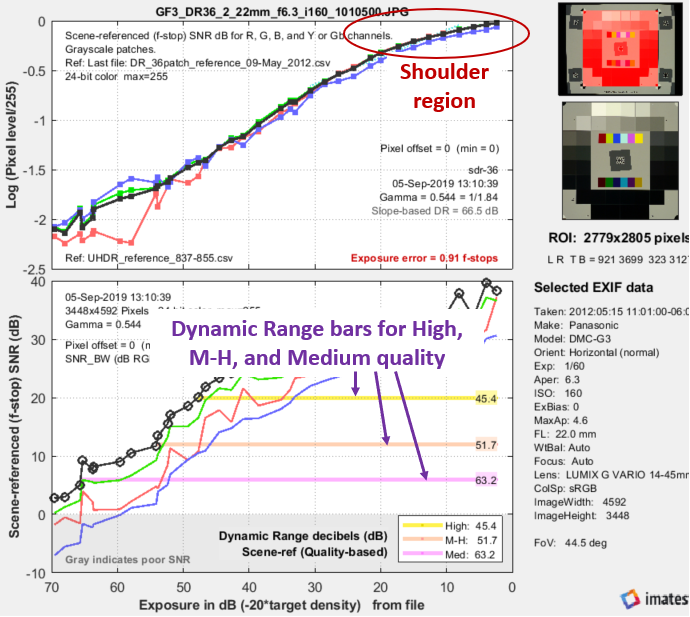Panasonic G3, ISO 160, in-camera JPEG, run with Color/Tone Auto (Multitest). Note the “shoulder.”
The horizontal bars in the lower plot show the range of exposure for SNRscene-ref = 20, 12, and 6dB.

#### The human vision perspective: F-stop noise and scene (or scene-referenced) SNRThe human eye responds to relative luminance differences. That’s why we think of exposure in terms of zonesf-stops, or EV (exposure value), where a change of one unit corresponds to a factor of 2 change in exposure. The eye’s relative sensitivity is expressed by the Weber-Fechner law,

Δ≈ 0.01 L  –or–  ΔL/L ≈ 0.01

where ΔL is the smallest luminance difference the eye can distinguish. This equation is approximate. Effective ΔL tends to be larger in dark areas of scenes and prints due to visual interference (flare light) from bright areas.

When light is encoded by a camera into pixel levels, the scene contrast is usually altered, as explained in Gamma, Tonal Response, and related concepts. Low contrast encoding would tend to have lower noise (and better Signal-to-Noise Ratio, SNR) than higher contrast cameras. Because dynamic range is based on the scene, we need to remove the camera’s encoding. The result is called scene-referenced noise or SNR with units proportional to the luminance level.

Expressing noise in relative luminance units, such as f-stops, corresponds more closely to the eye’s response than standard pixel or voltage units. Noise in f-stops = Nf-stop is obtained by dividing the noise in pixel level units by the number of pixel levels per f-stop. (We use “f-stop” rather than “zone” or “EV” out of habit; any are OK.) Note that 1 f-stop = 0.301 Optical density units = 6.02dB (decibels) = log2(luminance).

Nf-stop is the scene noise in (logarithmic) units of f-stops, and must be distinguished from linear scene noise, Nscene, which has the same linear units as scene luminance Lscene. For signal in pixels = S,

 $$\displaystyle \text{F-stop noise } = N_{f-stop} = \frac{N_{pixels}}{dS/d(\text{f-stop})} = \frac{N_{pixels}}{dS/d(\log_2 ( L_{scene})}$$      $$\displaystyle\text{Using }\ \frac{d(\log_a(x))}{dx} = \frac{1}{x \ln (a)} \ ; \ \ \ \ \ d(\log_a(x)) = \frac{dx}{x \ln(a)}$$      $$\displaystyle N_{f-stop} = \frac{N_{pixels}}{dS/dL_{scene} \times \ln(2) \times L_{scene}} ≅ \frac{N_{pixels}}{dS/dL_{scene} \times L_{scene}}$$ where Npixels is the measured noise in pixels and $$d(\text{pixel})/d(\text{f-stop})$$ is the derivative of the signal (pixel level) with respect to scene luminance (exposure) measured in f-stops = log2(luminance).  ln(2) = 0.6931 has been dropped to maintain backwards compatibility with older Imatest calculations. Noting that luminance (exposure) is the signal level of the scene,      $$\displaystyle \text{Scene noise} = N_{scene} = \frac{N_{pixels}}{dS/dL_{scene}} ≅ N_{f-stop} \times L_{scene}$$  The key to these calculations is that the scene-referenced Signal-to-Noise Ratio, calculated from the measured signal S and noise Npixels must be the same as the scene SNR, which is based on Nscene, which cannot be measured directly.      $$\displaystyle \text{Scene Signal-to-Noise Ratio} = SNR_{scene} = \frac{L_{scene}}{N_{scene}} = \frac{1}{N_{f-stop}} = \text{Scene-referenced SNR} = SNR_{scene-ref}$$ the equation for Scene-referenced noise, $$N_{scene-ref}$$, which enables $$SNR_{scene-ref} = SNR_{scene}$$ to be calculated directly from $$S/N_{pixels}$$  is given above. Displays in Stepchart, Color/Tone Interactive, and Color/Tone Auto offer a choice between f-stop noise or Scene-referenced SNR (expressed as a ratio or in dB). Note that SNRscene-ref decreases as the slope of the tonal response curve decreases (often the result of flare light in dark patches).

The above-right image illustrates how the pixel spacing between f-stops (and hence d(pixel)/d(f-stop)) decreases with decreasing brightness. This causes f-stop noise to increase with decreasing brightness, visible in the figures above.

Since f-stop noise and scene-referenced SNR are functions of scene luminance, largely independent of image signal processing and fogging from flare light, they are an excellent indicators of real-world camera performance. They are the basis of Imatest Dynamic Range measurements.

# Logarithmic wedges: a superior design

We introduce the logarithmic wedge pattern, which has several advantages over the widely-used hyperbolic wedges found in ISO 12233 (current and older) and eSFR ISO charts. Logarithmic wedges will be available in eSFR ISO charts starting in 2022, and will be supported by Imatest 2021.2+.

The key advantage of logarithmic wedges is that charts with large fmax/fmin ratios work well for systems with a wide range of resolutions, unlike hyperbolic wedges, where large ratios cause high frequencies occupy excessive real estate and low frequencies to become highly compressed. The fmax/fmin ratio of the hyperbolic wedges in the ISO 12233/2017 chart is only 12.5:1 — insufficient for modern high resolution cameras.

# Gamma, Tonal Response Curve, and related concepts

### Introduction

In this post we discuss a number of concepts related to tonal response and gamma that are scattered around the Imatest website, making them hard to find. We’re putting them together here in anticipation of questions about gamma and related concepts, which keep arising.

Gamma (γ) is the average slope of the function that relates the logarithm of pixel levels (in an image file) to the logarithm of exposure (in the scene).

$$\text{log(pixel level)} \approx \gamma \ \text{log(exposure)}$$

This relationship is called the Tonal Response Curve, (It’s also called the OECF = Opto-Electrical Conversion Function). The average is typically taken over a range of pixel levels from light to dark gray.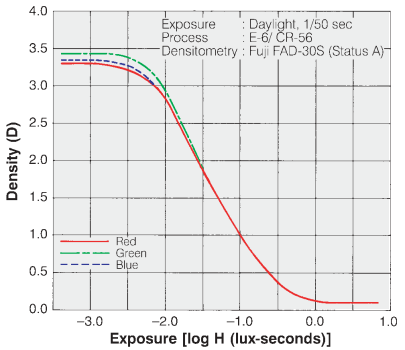Tonal Response Curve for Fujichrome Provia 100F film. The y-axis is reversed from the digital plots.

Tonal Response Curves (TRCs) have been around since the nineteenth century, when they were developed by Hurter and Driffield— a brilliant accomplishment because they lacked modern equipment. They are widely used for film. The Tonal Response Curve for Fujichrome Provia 100F film is shown on the right. Note that the y-axis is reversed from the digital plot shown below, where log(normalized pixel level) corresponds to $$D_{base} – D\text{, where } D_{base} = \text{base density} \approx 0.1$$.

Equivalently, gamma can be thought of as the exponent of the curve that relates pixel level to scene luminance.

$$\text{pixel level = (RAW pixel level)}^ \gamma \approx \text{exposure} ^ \gamma$$

There are actually two gammas: (1) encoding gamma, which relates scene luminance to image file pixel levels, and (2) display gamma, which relates image file pixel levels to display luminance. The above two equations (and most references to gamma on this page) refer to encoding gamma. The only exceptions are when display gamma is explicitly referenced, as in the Appendix on Monitor gamma.

The overall system contrast is the product of the encoding and decoding gammas. More generally, we think of gamma as contrast.

Encoding gamma is introduced in the image processing pipeline because the output of image sensors, which is linear for most standard (non-HDR) image sensors, is never gamma-encoded. Encoding gamma is typically measured from the tonal response curve, which can be obtained photographing a grayscale test chart and running Imatest’s Color/Tone module (or the legacy Stepchart and Colorcheck modules).

Display gamma is typically specified by the color space of the file. For the most common color space in Windows and the internet, sRGB, display gamma is approximately 2.2. (It actually consists of a linear segment followed by a gamma = 2.4 segment, which together approximate gamma = 2.2.) For virtually all computer systems, display gamma is set to correctly display images encoded in standard (gamma = 2.2) color spaces.

Here is an example of a tonal response curve for an in-camera JPEG for a high quality consumer camera, measured with Imatest Color/Tone.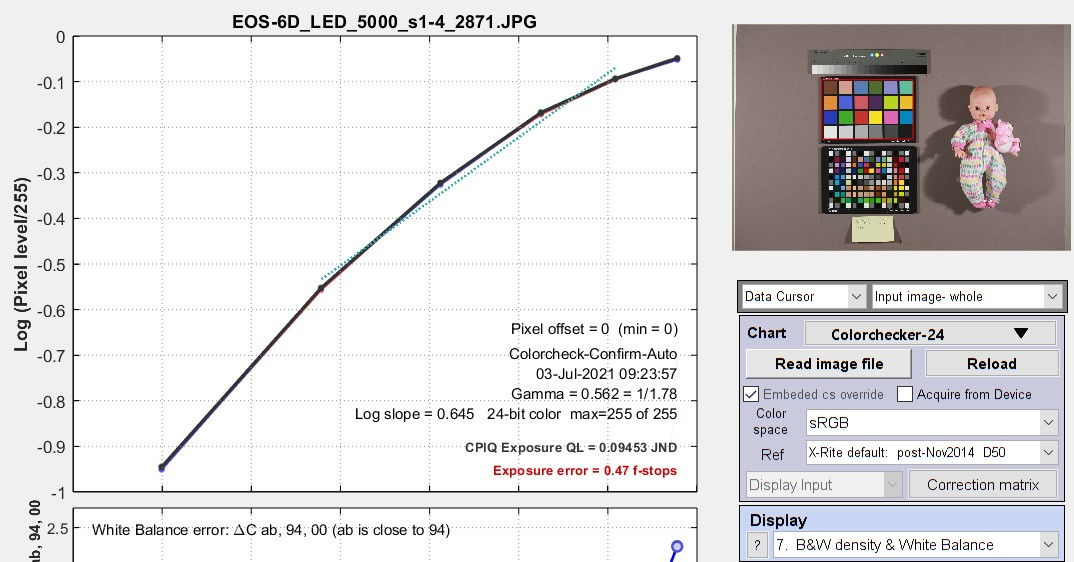Tonal response curve measured by the Imatest Color/Tone module
from the bottom row of the 24-patch Colorchecker,

Note that this curve is not a straight line. It’s slope is reduced on the right, for the brightest scene luminance. This area of reduced slope is called the “shoulder”. It improves the perceived quality of pictorial images (family snapshots, etc.) by reducing saturation or clipping (“burnout”) of highlights, thus making the response more “film-like”. A shoulder is plainly visible in the Fujichorme Provia 100D curve, above. Shoulders are almost universally applied in consumer cameras; they’re less common in medical or machine vision cameras.

Because the tonal response is not a straight line, gamma has to be derived from the average (mean) value of a portion of the tonal response curve.

### Why use logarithms?

Logarithmic curves have been used to express the relationship between illumination and response since the nineteenth century because the eye’s response to light is logarithmic. This is a result of the Weber-Fechner law, which states that the perceived change dp to a change dS in an initial stimulus S is

$$dp = dS/S$$

Applying a little math to this curve, we arrive at $$p = k \ln(S/S_0)$$  where ln is the natural logarithm (loge).

From G. Wyszecki & W. S. Stiles, “Color Science,” Wiley, 1982, pp. 567-570, the minimum light difference ΔL that can be perceived by the human eye is approximately

$$\Delta L / L = 0.01 = 1\%$$. This number may be too stringent for real scenes, where ΔL/L may be closer to 2%.

Advantages of gamma encoding  (Spoiler: It’s Dynamic Range.)

In photography, we often talk about zones (derived from Ansel Adams’ zone system). A zone is a range of illumination L that varies by a factor of two, i.e., if the lower and upper boundaries of a zone are L1 and L2, then L2 /L1 = 2 or log2(L2) – log2(L1) = 1.

 1 2 3 4 5 6 7 8 9

Zone system chart for gamma = 2.2 (sRGB color space, etc.)

The steps between each zone has equal visual weight, except for very dark zones where the differences are hard to see.

For a set of zones z = {1, 2, 3, ..} the relative illumination of zone n is 2-(z-1) = {1, 0.5, 0.25, 0.125, …}. The illumination difference between zones is {0.5, 0.25, 0.125, …}. The scene illumination ratio is always 2:1.

 Geek alert: a lot of numbers follow— all of which are intended to show how gamma encoding increases the effective dynamic range. The total number of available pixel levels B is a function of the bit depth, B = 2(bit depth). For bit depth = 8, B = 256; for bit depth = 16, B = 65536. The relative pixel level of zone n for encoding gamma γe is bn = B × 2-(n-1)γe.For linear gamma (γe = 1), bn= {1.0, 0.5, 0.25, 0.125, …} (the same as L). For γe= 1/2.2 = 0.4545, bn = {1.0000, 0.7297, 0.5325, 0.3886, 0.2836, 0.2069, 0.1510 ,0.1102, …}. The relative pixel levels bn decrease much more slowly than for γe = 1. The relative number of pixel levels in each zone is npγ(i) = bn(i) – bn(i+1), which brings us to the heart of the issue. For a maximum  pixel level of 2(bit depth)-1 = 255 for widely-used files with bit depth = 8 (24-bit color), the total number of pixels in each zone is Npγ(i) = 2(bit depth)npγ(i).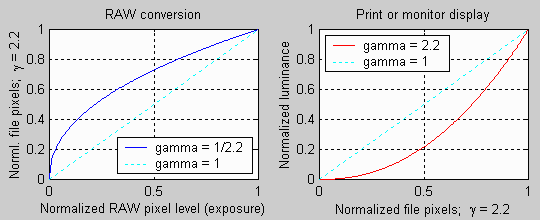When gamma = 2.2, the darker zones in the file contain more pixel levels. For linear images (γe = 1), npγ(i) = {0.5, 0.25, 0.125, 0.0625, …}, i.e., half the pixel levels would be in the first zone, a quarter would be in the second zone, etc. For files with bit depth = 8, the zones starting from the brightest would have Npγ(i) = {128, 64, 32, 16, 8, 4, 2, 1 …} pixel levels of 256 (0-255). By the time you reached the 5th or 6th zone, the spacing between pixel levels would be small enough to cause significant “banding”, limiting the dynamic range. For images encoded with γe = 1/2.2 = 0.4545, the relative number of pixels in each zone would be bn(i) = {0.2703, 0.1972, 0.1439, 0.1050, 0.0766, 0.0559, 0.0408, 0.0298, 0.0217, 0.0159…}, and the total number Npγ(i) would be {69.2, 50.5, 36.8, 26.9, 19.6, 14.3, 10.4, 7.63, 5.56, 4.06, …} of 256. The sixth zone, which has only 4 levels for γe = 1, has 14.3 levels. The 10th gamma-encoded zone has the same number of levels (4) as the 6th linear zone, i.e., an effective dynamic range increase of up to four zones (f-stops; factors of 2), i.e., These numbers show that gamma-encoding greatly improves the effective dynamic range of images with limited bit depth by making the distribution of pixel levels in the zones more uniform.  Of course, the improvement will be less if flare light in dark regions is the limiting factor.

### What gamma (and Tonal Response Curve) should I expect? And what is good?

JPEG images from consumer cameras typically have complex tonal response curves (with shoulders), with gamma (average slope) in the range of 0.45 to 0.6. This varies considerably for different manufacturers and models. The shoulder on the tonal response curve allows the the slope in the middle tones to be increased without worsening highlight saturation. This increases the apparent visual contrast, resulting in “snappier” more pleasing images.

RAW images from consumer cameras have to be decoded using LibRaw/dcraw. Their gamma depends on the Output gamma and Output color space settings (display gamma is shown in the settings window). Typical results are

• Encoding gamma ≅ 0.4545 with a straight line TRC (no shoulder) if conversion to a color space (usually sRGB or Adobe RGB) is selected;
• Encoding gamma ≅ 1.0 if a minimally processed image is selected.

RAW images from binary files (usually from development systems) have straight line gamma of 1.0, unless the Read Raw Gamma setting (which defaults to 1) is set to a different value.

Flare light can reduce measured gamma by fogging shadows, flattening the Tonal Response Curve for dark regions. Care should be taken to minimize flare light when measuring gamma.

That said, we often see values of gamma that differ significantly from the expected values of ≅0.45-0.6 (for color space images) or 1.0 (for raw images without gamma-encoding). It’s difficult to know why without a hands-on examination of the system. Perhaps the images are intended for special proprietary purposes (for example, for making low contrast documents more legible by increasing gamma); perhaps there is a software bug.

### Gamma and MTF measurement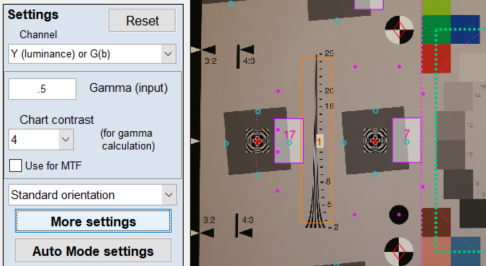MTF (Modulation Transfer Function, which is equivalent to Spatial Frequency Response), which is used to quantify image sharpness, is calculated assuming that the signal is linear. For this reason, gamma-encoded files must be linearized, i.e., the gamma encoding must be removed. The linearization doesn’t have to be perfect, i.e., it doesn’t have be the exact inverse of the tonal response curve. For most images (especially where the chart contrast is not too high), a reasonable estimate of gamma is sufficient for stable, reliable MTF measurements. The settings window for most MTF calculation has a box for entering gamma (or setting gamma to be calculated from the chart contrast).

Gamma is entered in the Setup or More settings window for each MTF module. They are described in the documentation for individual Rescharts modules. For Slanted-edge modules, they appear in the Setup window (crop shown on the right) and in the More settings window (crop shown below).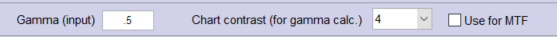Gamma (input) defaults to 0.5, which is a reasonable approximation for color space files (sRGB, etc.), but is incorrect for raw files, where gamma ≅ 1. Where possible we recommend entering a measurement-based estimate of gamma.

#### Determine gamma from a grayscale pattern

Gamma is often measured from grayscale patterns, which can be in separate charts or included in any of several sharpness charts— SFRplus, eSFR ISO, Star, Log F-Contrast, and Random (but not Checkerboard). The grayscale pattern from the eSFR ISO and SFRplus chart is particularly interesting because it shows the levels of the light and dark portions of the slanted-edge patters used to measure MTF.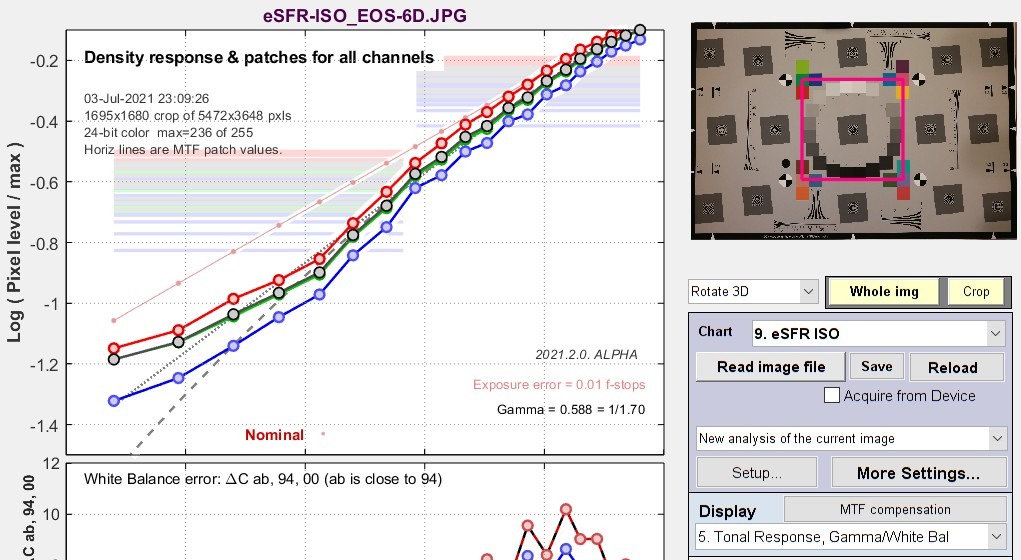Tonal response plot from eSFR ISO chart

Gamma = 0.588 here: close to the value (shown above) measured from the X-Rite Colorchecker for the same camera. The interesting thing about this plot is the pale horizontal bars, which represent the pixel levels of the light and dark portions of the selected slanted-edge ROIs (Regions of Interest). This lines let you see if the slanted-edge regions are saturating or clipping. This image shows that there will be no issue.

#### Determine gamma from each individual slanted-edge

Enter chart contrast and check Use for MTF. Only available for slanted-edge MTF modules. When Use for MTF is checked, the gamma (input) box is disabled.

This setting uses the measured of contrast of flat areas P1 and P2 (light and dark portions of each individual slanted-edge Region of Interest (ROI), away from the edge itself) to calculate the gamma for each edge. It is easy to use and quite robust. The only requirement is that the printed chart contrast ratio be known and entered. (It is 4:1 or 10:1 for nearly all Imatest slanted-edge charts.) This method is not reliable for chart contrasts higher than 10:1.

$$\displaystyle gamma\_encoding = \frac{\log(P_1/P_2)}{\log(\text{chart contrast ratio)}}$$

 A brief history of chart contrast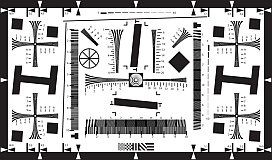The ISO 12233:2000 standard called for a chart contrast of at least 50:1. This turned out to be a poor choice: The high contrast made it difficult to avoid clipping (flattening of the tonal response curve for either the lightest or darkest areas), which exaggerates MTF measurements (making them look better than reality). There is no way to calculate gamma from the ISO 12233:2000 chart (shown on the right). This issue was finally corrected with ISO 12233:2014 (later revisions are relatively minor), which specifies a 4:1 edge contrast, which not only reduces the likelihood of clipping, but also makes the MTF calculation less sensitive to the value of gamma used for linearization. The old ISO 12233:2000 chart is still widely used: we don’t recommend it.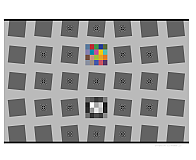The SFRplus chart, which was introduced in 2009, originally had an edge contrast of 10:1 (often with a small number of 2:1 edges). After 2014 the standard contrast was changed to 4:1 (shown on the right). The eSFR ISO chart (derived from the 2014 standard) always has 4:1 edge contrast. Both SFRplus and eSFR ISO have grayscales for calculating tonal response and gamma. SFRreg and Checkerboard charts are available in 10:1 and 4:1 contrast. Advantages of the new charts are detailed here and here. Imatest chrome-on-glass (CoG) charts have a 10:1 contrast ratio: the lowest that can be manufactured with CoG technology. Most other CoG charts have very high contrast (1000:1, and not well-controlled). We don’t recommend them. We can produce custom CoG charts quickly if needed.

### Which patches are used to calculate gamma?

This is an important question because gamma is not a “hard” measurement. Unless the Tonal Response Curve says pretty close to a straight line log pixel level vs. log exposure curve, its measured value depends on which measurement patches are chosen. Also, there have been infrequent minor adjustments to the patches used for Imatest gamma calculations— enough so customers occasionally ask about tiny discrepancies they find.

For the 24-patch Colorchecker, patches 2-5 in the bottom row are used (patches 20-23 in the chart as a whole).

For all other charts analyzed by Color/Tone or Stepchart, the luminance Yi for each patch i (typically 0.2125×R + 0.7154×G + 0.0721×B) is found, and the minimum value Ymin, maximum value Ymax, and range Yrange = YmaxYmin is calculated. Gamma is calculated from patches where Ymin + 0.2×Yrange  < Yi  < Ymax – 0.1×Yrange . This ensures that light through dark gray patches are included and that saturated patches are excluded.

### History: where did gamma ≅ 2.2 come from?

It came from the Cathode Ray Tubes (CRTs) that were universally used for television and video display before modern flat screens became universal. In CRTs, screen brightness was proportional to the control grid voltage raised to the 2 to 2.5 power. For this reason, signals had to be encoded with the approximate inverse of this value, and this encoding stuck. As we describe above, in “What is gained by applying a gamma curve?”, there is a real advantage to gamma encoding in image files with a limited bit depth, especially 8-bit files, which only have 256 possible pixel levels (0-255).

### Why are images converted from raw files often darker then JPEGs from the same capture?

Many cameras have the option of producing both interchangeable image files (almost always JPEG) and raw files from the same image capture. The raw-converted files are frequently darker— sometimes much darker— than the corresponding JPEGs. This is not an accident.

In Imatest, raw files are typically converted to interchangeable files (usually TIFF) using LibRaw, which has several presets and additional options. The most commonly-used presets are Color 24-bit sRGB (gamma ≅ 2.2) and Color 48-bit Adobe RGB (gamma = 2.2). These settings use EXIF metadata in the raw file to perform white balance and color correction (for the selected color space) and apply an appropriate straight-line gamma curve, $$\text{log(pixel level)} = \gamma \ \text{log(exposure)}$$ . The tonal response curve, displayed logarithmically, is a straight line with no “shoulder” or other curvature.

JPEGs, on the other hand, frequently have a “shoulder”— a region where gamma (contrast) is gradually reduced— in the highlights. The shoulder improves the perceived pictorial quality of the image by reducing saturation (or “burnout”) of the highlights. It was a part of film response curves, long taken for granted. In order to achieve this shoulder, the exposure has to be reduced below the optimum level for a straight gamma curve to allow some “headroom” for the shoulder (before highlights are saturated). Here is an example:  in-camera JPEG and LibRaw-converted raw images of the Colorchecker charts and doll, whose tonal response curve are shown above or the in-camera JPEG and below for the LibRaw-converted TIFF.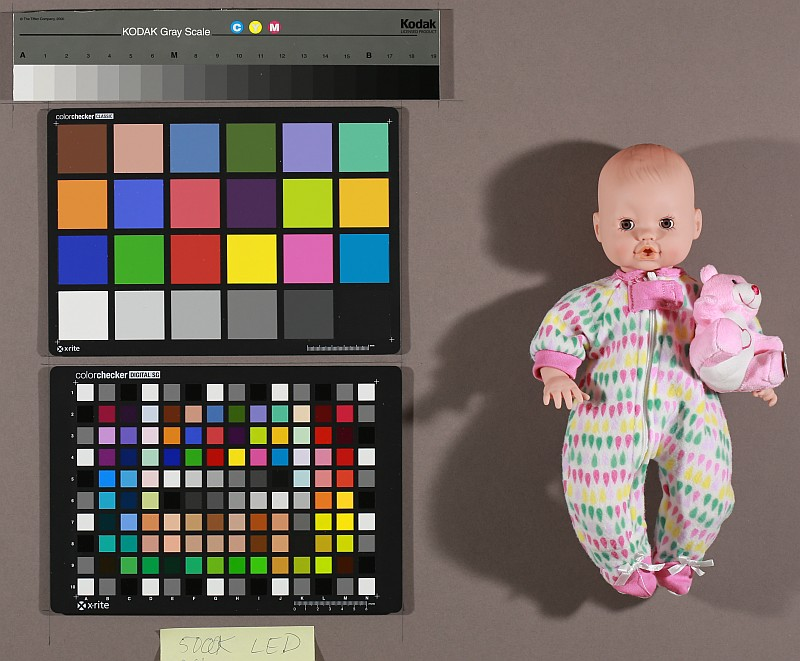in-camera JPEG. Tonal response curveabove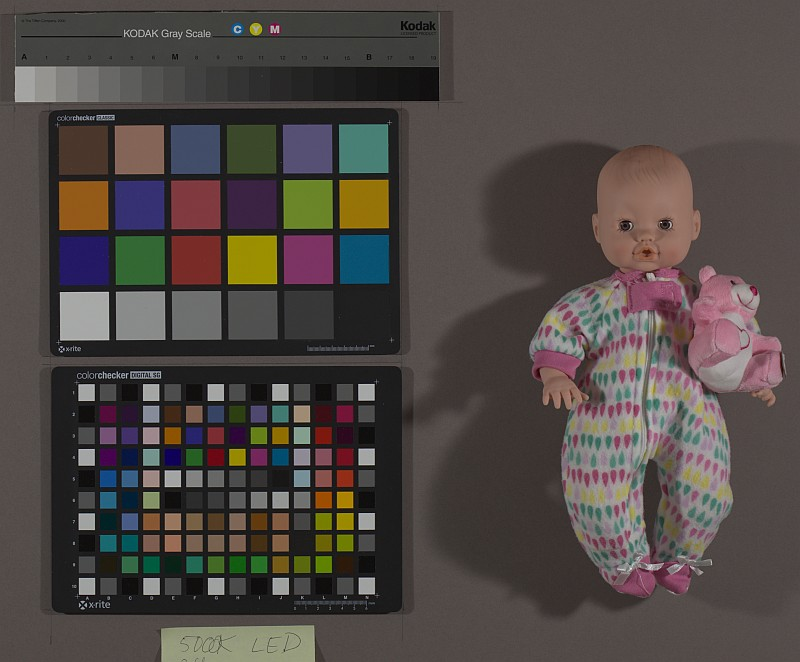converted from CR2 raw with LibRaw. Tonal response below

Here is the (nearly) straight-line density response for the converted CR2 raw image on the right. Though this image is typical, we have seen much larger differences between JPEG and converted raw files.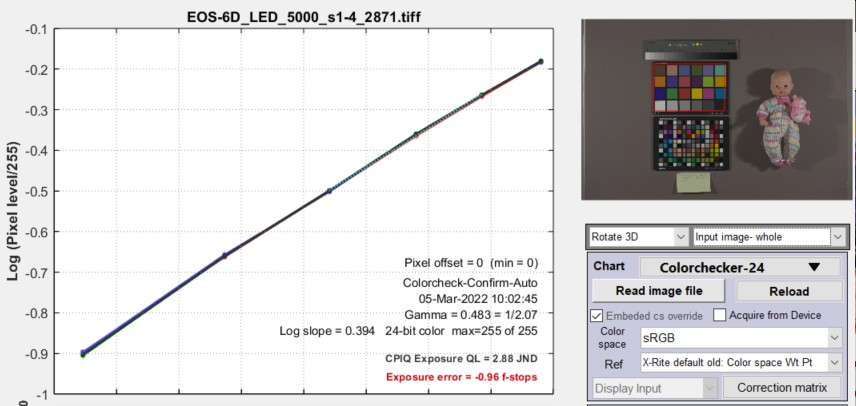Tonal response curve from the bottom row of the Colorchecker
from the image on the right, above (converted from raw with LibRaw)

### Tone mapping

Tone mapping  is a form of nonuniform image processing that lightens large dark areas of images to make features more visible. It reduces global contrast (measured over large areas) while maintaining local contrast (measured over small areas) so that High Dynamic Range (HDR) images can be rendered on displays with limited dynamic range. it can usually be recognized by extremely low values of gamma (<0.25; well under the typical values around 0.45 for color space images), measured with standard grayscale charts.

Tone mapping can seriously spoil gamma and Dynamic Range measurements, especially with standard grayscale charts. The Contrast Resolution chart was designed to give good results (for the visibility of small objects) in the presence of tone mapping, which is becoming increasingly popular with HDR images.

### Logarithmic encoding

Logarithmic encoding, which has a similar intent to gamma encoding, are widely used in cinema cameras. According to Wikipedia’s Log Profile page, every camera manufacturer has its own flavor of logarithmic color space. Since they are rarely, if ever, used for still cameras, Imatest does little with them apart from calculating the log slope (n1, below). A detailed encoding equation from renderstory.com/log-color-in-depth is $$f(x) = n_1 \log(r_1 n_3+ 1) + n_2$$.

From context, this can be expressed as

$$\text{pixel level} = p = n_1 \log(\text{exposure} \times n_3+ 1) + n_2$$

Since log(0) = -∞, exposure must be greater than 1/n3 for this equation to be valid, i.e., there is a minimum exposure value (that defines a maximum dynamic range).

By comparison, the comparable (simplified) equation for gamma encoding is

$$\log(\text{pixel level}) = \log(p) = \gamma \log(\text{exposure}) \ ; \ \ \ \ \ \ p = \text{exposure}^{\gamma}$$

The primary difference is that pixel level instead of log(pixel level) is used the equation. The only thing Imatest currently does with logarithmic encoding is to display the log slope n1, shown in some tonal response plots. Imatest could do more on customer request.

Logarithmic encoding has a very different appearance from gamma encoding when viewed in log-log density plots, as shown on the right. These curves are useful for identifying logarithmic encoding. The plot below is a density response curve, made with an Imatest dynamic range chart, for a camera that produces still and video output. At this time we’re not sure it’s intentionally logarithmic, but it certainly looks it. The flattened response and steps on the left are caused by flare light.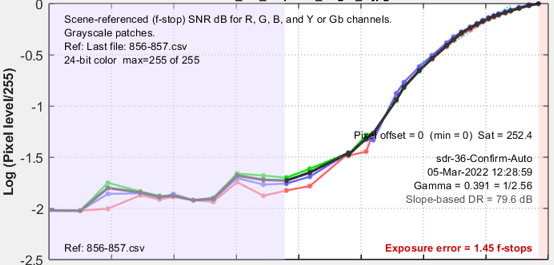Response of an MP4 frame from a camera that produces both still and video images.

Dynamic range limitation  An issue with logarithmic spaces is that their dynamic range is strictly limited by the math since log(0) = -∞. Using the above equation and assuming that log represents log10 and n2 = 1

$$p = \gamma \ \log_{10}(exp)+1$$

If we assume that exp is normalized to a maximum value of expmax = 1,  p(expmax) = p(1) = 1. The darkest level that can be reproduced is p(expmin) = γ log10(expmin)+1 = 0.

$$\displaystyle \log_{10}(exp_{min}) = -1/\gamma \ ; \ \ \ \ exp_{min} = 10^{-1/\gamma}$$

For gamma = 0.5 (shown in the plot, above right), expmin = 10-2 = 0.01, equivalent to

$$\text{Dynamic Range} = exp_{max}/exp_{min} = 1/0.01 = 100 = 40\text{dB}$$

#### Logarithmic response example

interceptor121.com/2021/02/07/the-truth-about-v-log/ has detailed equations for Panasonic V-LOG encoding. We programmed them because

1. it’s a real example and
2. it was easy.

The curve is a hybrid curve with a logarithmic section for illumination above cut1 = 0.01 and a linear section below cut1. In this respect it is somewhat similar to sRGB, which has linear and gamma sections that average to approximately gamma = 2.2.

The encoding is intended to improve dynamic range, but we have some doubts because the slope of the log-log plot is very low for the darkest regions (x < 10-3).

 This brings us to the big question: Why is logarithmic encoding widely used in video/cinema systems? Coming from still photography, where gamma encoding is widely used (but log encoding isn’t), we are aware that gamma encoding accomplishes the same goals without the dynamic range limitation. If you have a good answer, please

 Misinformation warning — The Internet is full of misinformation about logarithmic color spaces. A good example is the B&H page, Understanding Log-Format Recording, which says, ” If your video—like most video—is not recorded using a log picture profile, chances are the exposure is being recorded in a linear fashion.” This is completely incorrect.  The author seems to have no inkling that gamma encoding exists. Then he compounds the confusion with statements like “The problem arises with the realization that the exposure values with which we measure light are not linear. An exposure “stop” represents a doubling or halving of the actual light level, not an increase by some arbitrary linear value on a scale. You could, in fact, say exposure values are themselves logarithmic!”.  Exposure Value (EV) is a relative measurement unit based on factors 2 (log2(exposure level)) that corresponds to the sensitivity of the human eye. It has nothing to do with the camera itself.  For the record: Most digital sensors have a linear response, with more than 8 bits for high quality cameras (12, 14, or more bits are common). Following Analog-to-Digital conversion, most still cameras encode the image using a gamma curve. Video cameras use either gamma or log curves. As we mentioned above, this extends the effective dynamic range for gamma encoding.

### Appendix I: Monitor gamma

Monitors are not linear devices. They are designed so that Brightness is proportional to pixel level raised to the gamma power, i.e., $$\text{Brightness = (Pixel level)}^{\gamma\_display}$$.

For most monitors, gamma should be close to 2.2, which is the display gamma of the most common color spaces, sRGB (the Windows and internet standard) and Adobe RGB.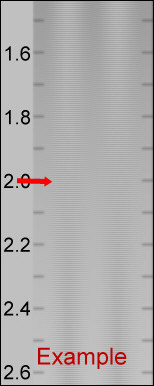The chart on the right is designed for a visual measurement of display gamma. But it  rarely displays correctly in web browsers. It has to be displayed in the monitor’s native resolution, 1 monitor pixel to 1 image pixel. Unfortunately, operating system scaling settings and browser magnifications can make it difficult. To view the gamma chart correctly, right-click on it, copy it, then paste it into Fast Stone Image Viewer. This worked well for me on my fussy system where the main laptop monitor and my ASUS monitor (used for these tests) have different scale factors (125% and 100%, respectively). The gamma will be the value on the scale where the gray area of the chart has an even visual density. For the (blurred) example on the left, gamma = 2. Although a full monitor calibration (which requires a spectrophotometer) is recommended for serious imaging work, good results can be obtained by adjusting the monitor gamma to the correct value. We won’t discuss the process in detail, except to note that we have had good luck with Windows systems using QuickGamma.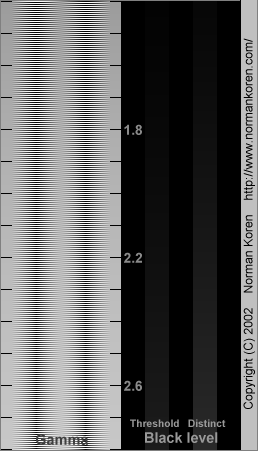Gamma chart. Best viewed with Fast Stone image viewer.

#### Appendix II: Tonal response, gamma, and related quantities

For completeness, we’ve updated and kept this table from elsewhere on the (challenging to navigate) Imatest website.

Parameter Definition
Tonal response curve The pixel response of a camera as a function of exposure. Usually expressed graphically as log pixel level vs. log exposure.
Gamma

Gamma is the average slope of log pixel levels as a function of log exposure for light through dark gray tones). For MTF calculations It is used to linearize the input data, i.e., to remove the gamma encoding applied by image processing so that MTF can be correctly calculated (using a Fourier transformation for slanted-edges, which requires a linear signal).

Gamma defaults to 0.5 = 1/2, which is typical of digital camera color spaces, but may be affected by image processing (including camera or RAW converter settings) and by flare light. Small errors in gamma have little effect on MTF measurements (a 10% error in gamma results in a 2.5% error in MTF50 for a normal contrast target). Gamma should be set to 0.45 or 0.5 when dcraw or LibRaw is used to convert RAW images into sRGB or a gamma=2.2 (Adobe RGB) color space. It is typically around 1 for converted raw images that haven’t had a gamma curve applied. If gamma  is set to less than 0.3 or greater than 0.8, the background will be changed to pink to indicate an unusual (possibly erroneous) selection.

If the chart contrast is known and is ≤10:1 (medium or low contrast), you can enter the contrast in the Chart contrast (for gamma calc.) box, then check the Use for MTF (√) checkbox. Gamma will be calculated from the chart and displayed in the Edge/MTF plot.

If chart contrast is not known you should measure gamma from a grayscale stepchart image. A grayscale is included in SFRplus, eSFR ISO and SFRreg Center ([ct]) charts. Gamma is calculated and displayed in the Tonal Response, Gamma/White Bal plot for these modules. Gamma can also be calculated from any grayscale stepchart by running Color/Tone Interactive, Color/Tone Auto, Colorcheck, or Stepchart. [A nominal value of gamma should be entered, even if the value of gamma derived from the chart (described above) is used to calculate MTF.]

 Gamma Gamma is the exponent of the equation that relates image file pixel level to luminance. For a monitor or print, $$\displaystyle \text{Output luminance = (pixel level)}^{gamma\_display}$$ When the raw output of the image sensor, which is typically linear, is converted to image file pixels for a standard color space, the approximate inverse of the above operation is applied. $$\displaystyle \text{Pixel level = (RAW pixel level)}^{gamma\_encoding} \approx exposure\ ^{gamma\_encoding}$$ This is equation is an approximation because the tonal response curve (which often has a “shoulder”— a region of decreased contrast in the highlights) doesn’t follow the gamma equation exactly. It is often a good approximation for light to dark gray tones: good enough for to reliably linearize the chart image if the edge contrast isn’t too high (4:1 is recommended in the ISO 12233:2014+ standard). $$\text{Total system contrast} = gamma\_encoding \times gamma\_display$$. The most common value of of display gamma is 2.2 for color spaces used in Windows and the internet, such as sRGB (the default) and Adobe RGB (1998). In practice, gamma is equivalent to contrast.  When the Use for MTF checkbox (to the right of the Chart contrast dropdown menu) is checked, camera gamma is estimated from the ratio of the light and dark pixel levels P1 and P2 in the slanted-edge region (away from the edge) if the chart contrast ratio (light/dark reflectivity) has been entered (and is 10:1 or less). Starting with $$P_1/P_2 = \text{(chart contrast ratio)}^{gamma\_encoding}$$, $$\displaystyle gamma\_encoding = \frac{\log(P_1/P_2)}{\log(\text{chart contrast ratio)}}$$

Shoulder A region of the tonal response near the highlights where the slope may roll off (be reduced) in order to avoid saturating (“bunring out”) highlights. Frequently found in pictorial images. Less common in machine vision images (medical, etc.) When a strong shoulder is present, the meaning of gamma is not clear.

# Comparing sharpness in cameras with different pixel count

### Introduction: The question

We frequently receive questions that go something like,

“How can you compare the sharpness of images taken with different cameras that have different resolutions (total pixel count) and physical pixel size (pitch or spacing)?”

The quick answer is that it depends on the application.

• Are you interested in the sharpness of the image over the whole sensor (typical of most pictorial photography— landscape, family, pets, etc.)? We call these applications image-centric.
• Do you need to measure details of specific objects (typical for medical imaging (we commonly work with endoscopes), machine vision, parts inspection, aerial reconnaissance, etc.)? We call these applications object-centric

In other words, what exactly do you want to measure?

where the objects can have very different pixel sizes.

The keys to appropriate comparison of different images are

• the selection of spatial frequency units for MTF/SFR (sharpness) measurements, and
• the selection of an appropriate summary metric (important since the most popular metric, MTF50, rewards software sharpening too strongly).

The table below is adapted from Sharpness – What is it, and how is it measured? We strongly recommend reviewing this page if you’re new to sharpness measurements.

 MTF Unit Application Equation Cycles/Pixel (C/P) Pixel-level measurement. Nyquist frequency fNyq is always 0.5 C/P. For comparing how well pixels are utilized. Not an indicator of overall image sharpness. Cycles/Distance (cycles/mm or cycles/inch) Cycles per physical distance on the sensor. Pixel spacing or pitch must be entered. Popular for comparing resolution in the old days of standard film formats (e.g., 24x36mm for 35mm film). For comparing Imatest results with output of lens design programs, which typically use cycles/mm. $$\frac{MTF(C/P)}{\text{pixel pitch}}$$ Line Widths/Picture Height (LW/PH) Measure overall image sharpness.  Line Widths is traditional for TV measurements. Note that 1 Cycle = 1 Line Pair (LP) = 2 Line Widths (LW). LW/PH and LP/PH are the best units for comparing the overall sharpness (on the image sensor) of cameras with different sensor sizes and pixel counts. Image-centric. note: for cropped images enter the original picture height into the more settings dimensions input $$2 \times MTF\bigl(\frac{LP}{PH}\bigr)$$ ; $$2 \times MTF\bigl(\frac{C}{P}\bigr) \times PH$$ Line Pairs/Picture Height (LP/PH) $$MTF\bigl(\frac{LW}{PH}\bigr) / 2$$ ; $$MTF\bigl(\frac{C}{P}\bigr) \times PH$$ Cycles/Angle: Cycles/milliradian Cycles/Degree Angular frequencies. Pixel spacing (pitch) must be entered. Focal length (FL) in mm is usually included in EXIF data in commercial image files. If it isn’t available it must be entered manually, typically in the EXIF parameters region at the bottom of the settings window. If pixel spacing or focal length is missing, units will default to Cycles/Pixel. Cycles/Angle (degrees or milliradians) is useful for comparing the ability of cameras to capture objects at a distance. For example, for birding (formerly called “birdwatching”) it is a good measure of a camera’s ability to capture an image of a bird at a long distance, independent of sensor and pixel size, etc. It is highly dependent on lens quality and focal length. Object-centric. It is also useful for comparing camera systems to the human eye, which has an MTF50 of roughly 20 Cycles/Degree (depending on the individual’s eyesight and illumination). $$0.001 \times MTF\bigl(\frac{\text{cycles}}{\text{mm}}\bigr) \times FL(\text{mm})$$ $$\frac{\pi}{180} \times MTF\bigl(\frac{\text{cycles}}{\text{mm}}\bigr) \times FL(\text{mm})$$ FL can be estimated from the simple lens equation, 1/FL=1/s1+1/s2, where s1 is the lens-to-chart distance, s2 is the lens-to-sensor distance, and magnification M=s2/s1. FL=s1/(1+1/|M|) = s2/(1+|M|) This equation may not give an accurate value of M because lenses can deviate significantly from the simple lens equation. Cycles/object distance: Cycles/object mm Cycles/object in Cycles per distance on the object being photographed (what many people think of as the subject). Pixel spacing and magnification must be entered. Important when the system specification references the object being photographed. Cycles/distance is useful for machine vision tasks, for example, where a surface is being inspected for fine cracks, and cracks of a certain width need to be detected. Object-centric. $$MTF\bigl( \frac{\text{Cycles}}{\text{Distance}} \bigr) \times |\text{Magnification}|$$ Line Widths/Crop Height Line Pairs/Crop Height Primarily used for testing when the active chart height (rather than the total image height) is significant. Not recommended for comparisons because the measurement is dependent on the (ROI) crop height. Line Widths/Feature Height (Px) Line Pairs/Feature Height (Px) (formerly Line Widths or Line Pairs/N Pixels (PH)) When either of these is selected, a Feature Ht pixels box appears to the right of the MTF plot units (sometimes used for Magnification) that lets you enter a feature height in pixels, which could be the height of a monitor under test, a test chart, or the active field of view in an image that has an inactive area. The feature height in pixels must be measured individually in each camera image. Example below. Useful for comparing the resolution of specific objects for cameras with different image or pixel sizes. Object-centric. $$2 \times MTF\bigl(\frac{C}{P}\bigr) \times \text{Feature Height}$$ $$MTF\bigl(\frac{C}{P}\bigr) \times \text{Feature Height}$$ PH = Picture Height in pixels. FL(mm) = Lens focal length in mm.  Pixel pitch = distance per pixel on the sensor = 1/(pixels per distance).   Note: Different units scale differently with image sensor and pixel size.

 Summary Metric Description Comments MTF50 MTFnn Spatial frequency where MTF is 50% (nn%) of the low (0) frequency MTF. MTF50 (nn = 50) is widely used because it corresponds to bandwidth (the half-power frequency) in electrical engineering. The most common summary metric; correlates well with perceived sharpness. Increases with increasing software sharpening; may be misleading because it “rewards” excessive sharpening, which results in visible and possibly annoying “halos” at edges. MTF50P MTFnnP Spatial frequency where MTF is 50% (nn%) of the peak MTF. Identical to MTF50 for low to moderate software sharpening, but lower than MTF50 when there is a software sharpening peak (maximum MTF > 1). Much less sensitive to software sharpening than MTF50 (as shown in a paper we presented at Electronic Imaging 2020). All in all, a better metric. MTF area normalized Area under an MTF curve (below the Nyquist frequency), normalized to its peak value (1 at f = 0 when there is little or no sharpening, but the peak may be » 1 for strong sharpening). A particularly interesting new metric because it closely tracks MTF50 for little or no sharpening, but does not increase for strong oversharpening; i.e., it does not reward excessive sharpening. Still relatively unfamiliar. Described in Slanted-Edge MTF measurement consistency. MTF10, MTF10P, MTF20, MTF20P Spatial frequencies where MTF is 10 or 20% of the zero frequency or peak MTF These numbers are of interest because they are comparable to the “vanishing resolution” (Rayleigh limit). Noise can strongly affect results at the 10% levels or lower. MTF20 (or MTF20P) in Line Widths per Picture Height (LW/PH) is closest to analog TV Lines. Details on measuring monitor TV lines are found here.

Although MTF50 (the spatial frequency where MTF falls to half its low frequency value) is the best known summary metric, we don’t recommend it because it rewards overly sharpened images too strongly. MTF50P is better in this regard, and MTF area normalized may be even better (though it’s not familiar or widely used). Summary metrics are described in Correcting Misleading Image Quality Measurements, which links to a paper we presented at Electronic Imaging 2020.

### Sharpening:  usually improves image appearance, but complicates camera comparisons

Sharpening, which is applied after image capture, either in the camera or in post-processing software, improves the visual appearance of images (unless it’s overdone), but makes camera comparisons difficult. It is described in detail here. Here are a few considerations.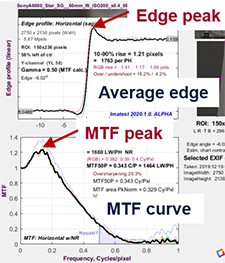Sharpening varies tremendously from camera to camera. Images converted from raw using Imatest’s Read Raw, dcraw, or LibRaw are unsharpened. JPEG images from high quality consumer cameras typically have moderate amounts of sharpening, characterized by limited edge overshoot (visible as halos) and a small bump in the MTF response. But all bets are off with camera phones and other mobile devices. We have seen ridiculous amounts of sharpening— with huge halos and MTF response peaks, which may make images look good when viewed on tiny screens, but generally wrecks havoc with image quality.

Sharpening can be recognized by the shape of the edge and the MTF response. Unsharpened images have monotonically decreasing MTF. Camera B below is typical. Sharpened images, illustrated on the right (from Correcting Misleading Image Quality Measurements) have bumps or peaks on both the edge and and MTF response. The edge on the right could be characterized as strongly, but not excessively, sharpened.

Sharpening summary — Since different amounts of sharpening can make comparisons between images difficult, you should examine the Edge/MTF plot for visible signs of sharpening. If possible, sharpening should be similar on different cameras. If this isn’t possible, a careful choice of the summary metric may be beneficial. MTF50P or MTF Area Peak Normalized are recommended.

### Example: A medical imaging system for measuring sharpness on objects of a specified size

Here is an example using the Rezchecker chart, whose overall size is 1-7/8” high x 1-5/8” wide. (The exact size isn’t really relevant to comparisons between cameras.)

The customer wanted to compare two very different cameras to be used for a medical application that requires a high quality image of an object over a specified field of view, i.e., the application is object-centric.

A Rezchecker chart was photographed with each camera. Here are the images. These images can be analyzed in either the fixed SFR module (which can analyze MTF in multiple edges) or in Rescharts Slanted-edge SFR (which only works with single edges).

Because we don’t know the physical pixel size of these cameras (it can be found in sensor spec sheets; it’s not usually in the EXIF data), we choose units Line Widths per Feature Height. When pixel size is known, line pairs per Object mm may be preferred (it’s more standard and facilitates comparisons). Here is the settings window from Rescharts Slanted-edge SFR.

The optional Secondary Readouts (obscured by the dropdown menu) are MTF50P and MTF Area PkNorm (normalized to the peak value). Feature Height (114 pixels for camera A; 243 for camera B) has to be measured individually for each image: easy enough in any image editor (I drew a rectangle in the Imatest Image Statistics module and used the height). Entering the feature height for each image is somewhat inconvenient, which is why Cycles/Object mm is preferred (if the sensor pixel pitch is known).

#### Results of the comparison

The Regions of interest (ROIs) are smaller than optimum, especially for camera A, where the ROI was only 15×28 pixels: well below the recommended minimum. This definitely compromises measurement accuracy, but the measurement is still good enough for comparing the two cameras.

Since there is no obvious sharpening (recognized by “halos” near edges; a bump or peak in the MTF response), the standard summary metrics are equivalent. MTF50 only fails when there is strong sharpening.

For resolving detail in the Rezchecker, camera B wins. At MTF50P = 54.8 LW/Feature Height, it is 20% better than camera A, which had MTF50P = 45.8 LW/Feature Height. Details are in the Appendix, below.

### Summary

 To compare cameras with very different specifications (pixel size; pixel pitch; field of view, etc.) the image needs to be categorized by task (or application). We define two very broad types of task. image-centric, where the sharpness of the image over the whole sensor is what matters. This is typical of nearly all pictorial photography— landscape, family, pets, etc. Line Widths (or Pairs) per Picture Height is the best MTF unit for comparing cameras.  object-centric,  where the details of specific objects (elements of the scent) are what matters. This is typical for medical imaging (for example, endoscopes), machine vision, parts inspection, aerial reconnaissance, etc. Bird and wildlife photography tends to be predominantly object-centric. Line Widths (or Pairs) per object distance or Feature Height are the appropriate MTF units for comparing object detail.  Sharpening complicates the comparison. Look carefully at the Edge/MTF plot for visible or measured signs of sharpening. The key sharpening measurement is overshoot. If possible, images should have similar amounts of sharpening. MTF50P or MTF Area Peak Normalized are recommended summary metrics. MTF50 is not recommended because it is overly sensitive to software sharpening and may therefore be a poor representation of the system’s intrinsic sharpness. This is explained in Correcting Misleading Image Quality Measurements.

# Three optical centers

Customers frequently want to know how we measure the optical center of the image (not to be confused with the geometrical center).  They may be surprised that we measure three, shown below in the 2D contour map, which is one of the options in Rescharts slanted-edge modules 3D & contour plots.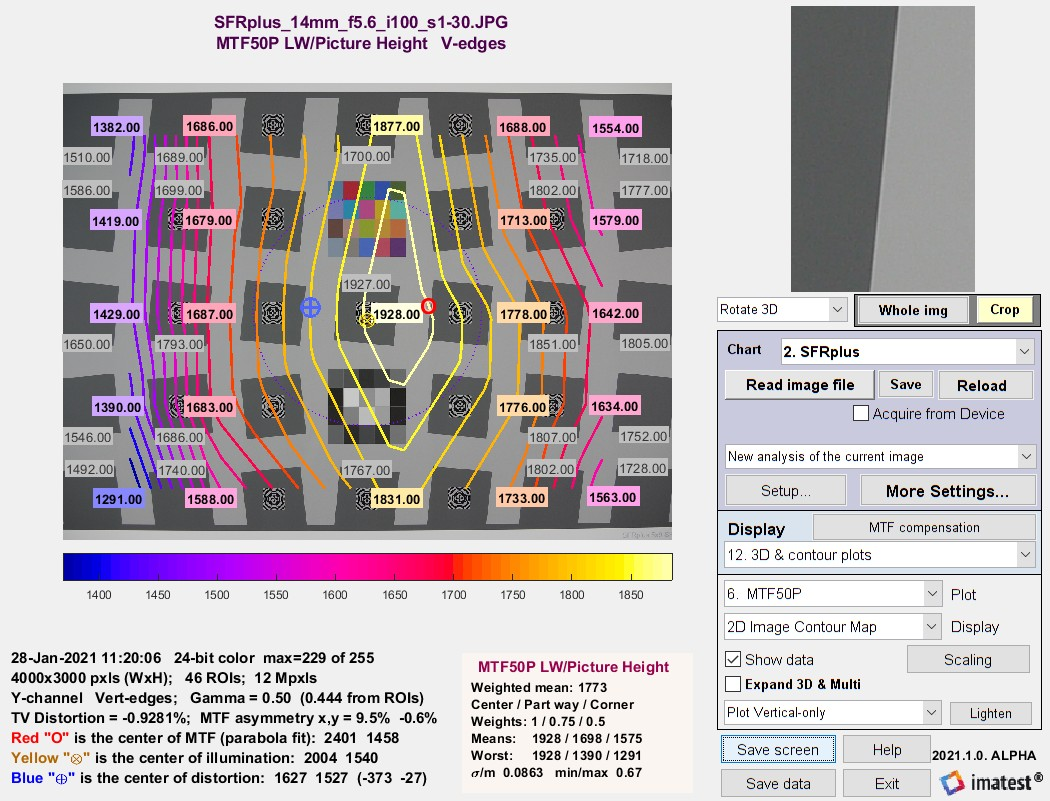SFRplus contour plot for MTF50P, showing three optical centers: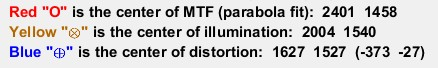For a normal well-constructed lens, the optical centers are all close to the geometric center of the image. They can diverge for defective or poorly-constructed lenses.

### Center of MTF (Center of Sharpness)

The center of MTF (or Sharpness) is the approximate location of maximum MTF, based on a second-order fit to results for slanted-edge charts (SFRplus, eSFR ISO, Checkerboard, and SFRreg). It is displayed (along with the two other optical centers) displayed in the 2D image contour map, which is one of the options in 3D & Contour plots

Algorithm:  Starting with an array of all MTF50 values and arrays for the x and y-locations of each value (the center of the regions), fit the MTF50 value to a second-order curve (a parabola). For the x (horizontal) direction,

MTF = axx2 + bxx + cx .

The peak location for this parabola, i.e., the

x-Center of MTF = xpeak = –bx /(2ax) .

It is reported if it’s inside the image. (It is not uncommon for it to be outside the image for tilted or defective lenses.) The y-center is calculated in exactly the same way.

MTF asymmetry is calculated from the same data and parabola fits as the Center of MTF. For the x- direction,

MTF50 (x-aysmmetry) = (MTFfit(R) − MTFfit(L)) / (MTFfit(R) + MTFfit(L))

where MTFfit(R) and MTFfit(L) are the parabola fits to MTF at the left and right borders of the image, respectively. MTF50 (y-asymmetry) is calculated with the same method.

### Center of illumination

The center of illumination is the brightest location in the image. It is usually not distinct, since brightness typically falls off very slowly from the peak location. It only represents the lens for very even back-illumination (we recommend one of our uniform light sources or an integrating sphere). For front illumination (reflective charts) it represents the complete system, including the lighting.

It is calculated very differently in Uniformity and Uniformity Interactive than it is in the slanted-edge modules.

Algorithm for Uniformity and Uniformity Interactive:  The location of the peak pixel (even if it’s smoothed) is not used because the result would be overly sensitive to noise. Find all points where the luminance channel g(x,y) (typically 0.2125*R + 0.7154*G + 0.0721*B) is above 95% of the maximum value. The x-center of illumination is the centroid of these values. The y-center is similar. This calculation is much more stable and robust than using peak values.

Cx = ∫x g(x)dx / ∫g(x)dx

Algorithm for Rescharts slanted-edge modules:  Starting with Imatest 2020.2, the mean ROI level of each region is calculated and can be plotted in 3D & contour plotsSFRplus contour plot for mean ROI levels, used to calculate
Center of Illumination ()

The calculation is identical to the Center of MTF calculation (above), except that mean ROI level is used instead of MTF50.

### Center of distortion

The center of distortion is the point around which Imatest distortion models assume that distortion is radially symmetric. It is calculated in Checkerboard, SFRplus, and Distortion (the older legacy module) when a checkbox for calculating it is checked. It is calculated using nonlinear optimization. If it’s not checked (or the center is not calculated), the geometrical center of the image is assumed to be the center of distortion.

Distortion measurements are described in Distortion: Methods and Modules.

What is the center of the image?  by R.G. Wilson and S. A. Shafer. Fifteen, count ’em fifteen optical centers. Do I hear a bid for sixteen? Going… going…

# Real-time focusing with Imatest Master direct data acquisition

Speed up your testing with real-time focusing in Imatest Master 2020.2.

Recent speed improvements allow for real-time focusing and allow users to analyze images from two types of sources:

Although the majority of images traditionally analyzed by Imatest have been from files (JPG, PNG, etc.), three modules, which can perform a majority of Imatest’s analyses, support direct data acquisition, and can be used for realtime analysis.

# Making Dynamic Range Measurements Robust Against Flare Light

### Introduction

A camera’s Dynamic Range (DR) is the range of tones in a scene that can be reproduced with adequate contrast and good signal-to-noise ratio (SNR). Camera DR is often limited by flare light, which is stray light in the image, primarily caused by reflections between lens elements. Flare light reduces DR by fogging images; i.e., washing out detail in dark areas. (more…)

# Correcting nonuniformity in slanted-edge MTF measurements

Slanted-edge regions can often have non-uniformity across them. This could be caused by uneven illumination, lens falloff, and photoresponse nonuniformity (PRNU) of the sensor.

Uncorrected nonuniformity in a slanted-edge region of interest can lead to an irregularity in MTF at low spatial frequencies. This disrupts the low-frequency reference which used to normalize the MTF curve. If the direction of the nonuniformity goes against the slanted edge transition from light to dark, MTF increases. If the nonuniformity goes in the same direction as the transition from light to dark, MTF decreases.

To demonstrate this effect, we start with a simulated uniform slanted edge with some blur applied.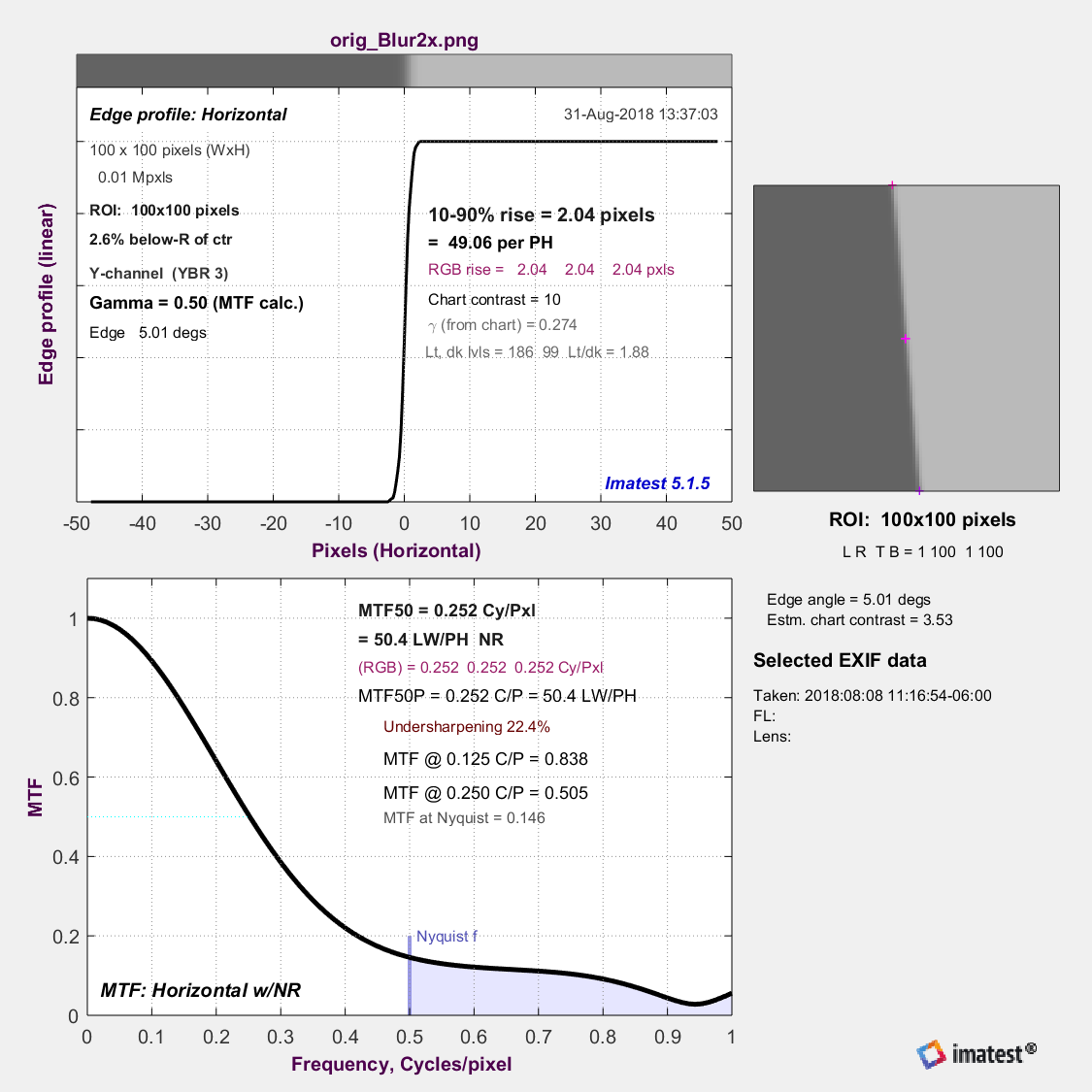Then we apply a simulated nonuniformity to the edge at different angles relative to the edge. This is modeled to match a severe case of nonuniformity reported by one of our customers: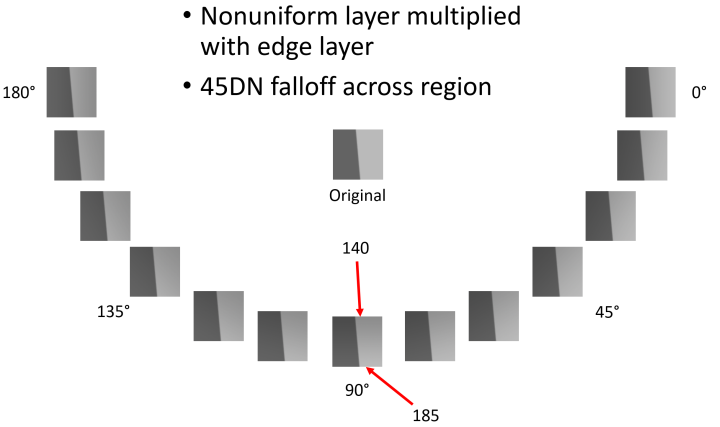Here is the MTF obtained from the nonuniform slanted edges: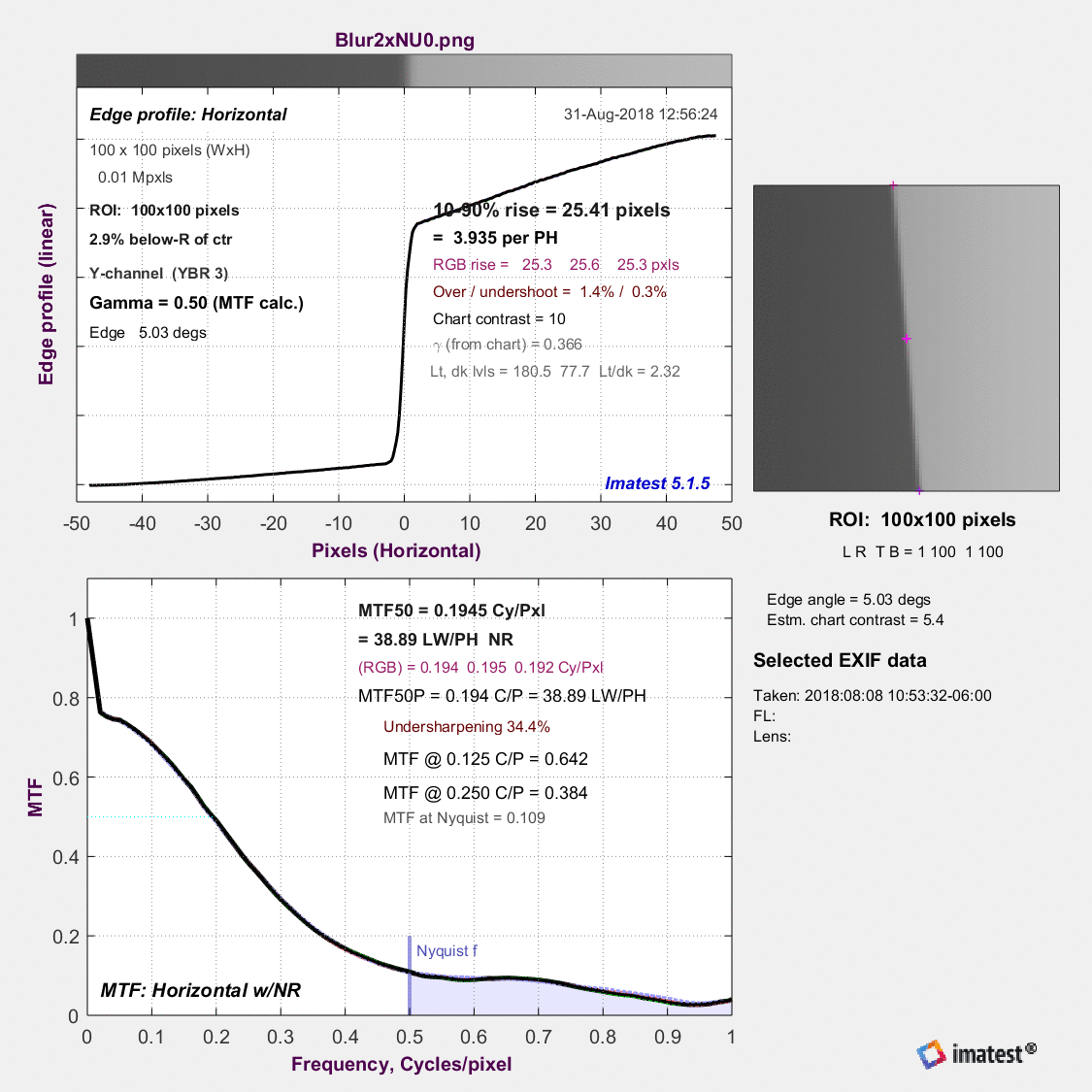If the nonuniformity includes an angular component that is parallel to the edge, this adds a sawtooth pattern to the spatial domain, which manifests as high-frequency spikes in the frequency domain. This is caused by the binning algorithm which projects brighter or darker parts of the ROI into alternating bins.

### Compensating for the effects of nonuniformity

Although every effort should be made to achieve even illumination, it’s not always possible (for example, in medical endoscopes and wide-FoV lenses).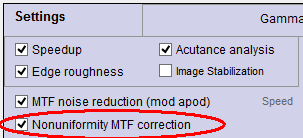Imatest 4.5+ has an option for dealing with this problem for all slanted-edge modules (SFR and Rescharts/fixed modules SFRplus, eSFR ISO, SFRreg, and Checkerboard). It is applied by checking the “Nonuniformity MTF correction” checkbox in the settings (or “More” settings) window, shown on the right.

When this box is checked, a portion of the spatial curve on the light side of the transition (displayed on the right in Imatest) is used to estimate the nonuniformity. The light side is chosen because it has a much better Signal-to-Noise Ratio than the dark side. In the above image, this would be the portion of the the edge profile more than about 6 pixels from the center. Imatest finds the first-order fit to the curve in this region, limits the fit so it doesn’t drop below zero, then divides the average edge by the first-order fit.

The applied compensation flattens the response across the edge function and significantly improves the stability of the MTF: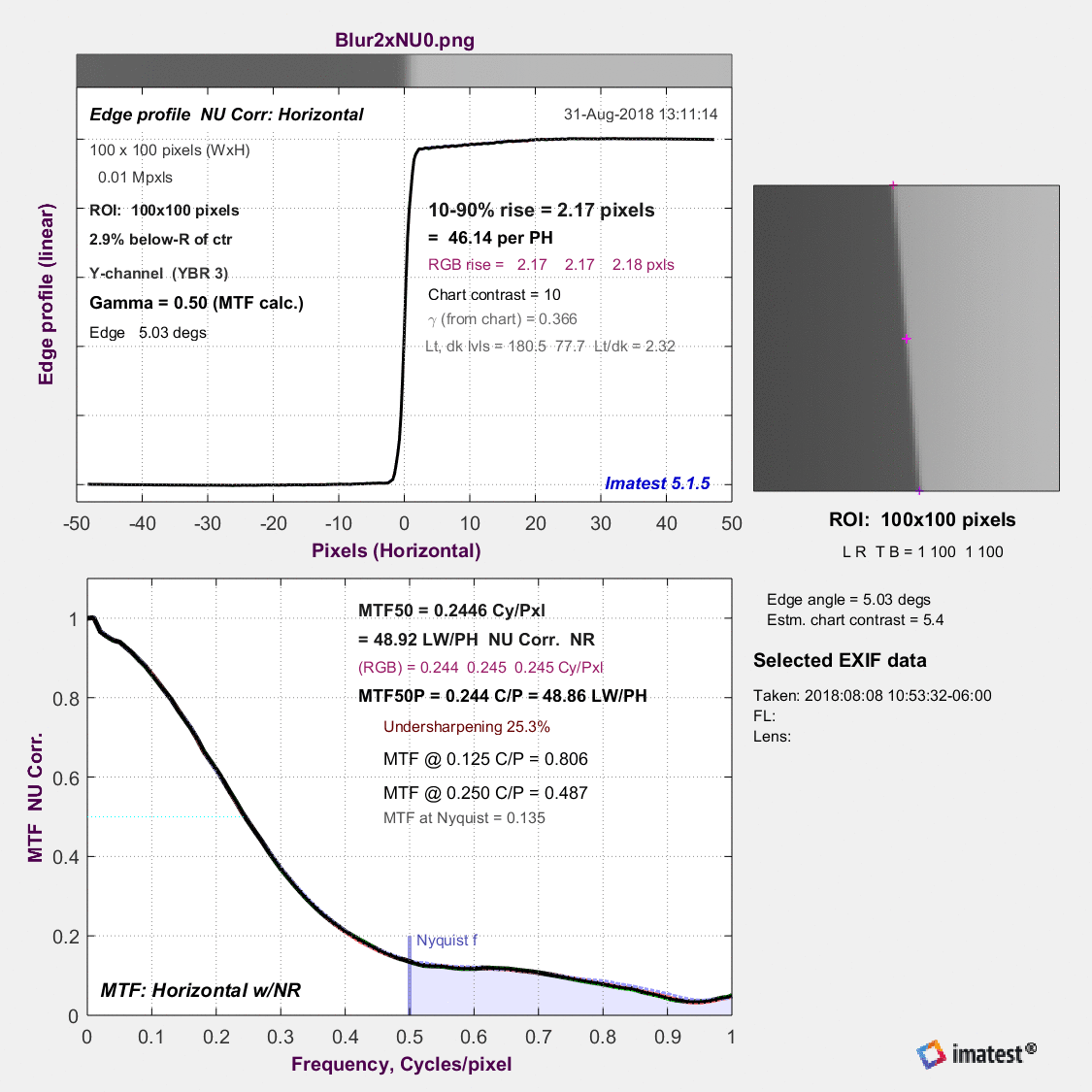### Summary

For this example, Imatest’s nonuniformity correction reduces our example’s -26.0% to +22.8% change in MTF down to a -3.5% to +4.7% change. This is an 83% reduction in the effect of the worst cases of nonuniformity.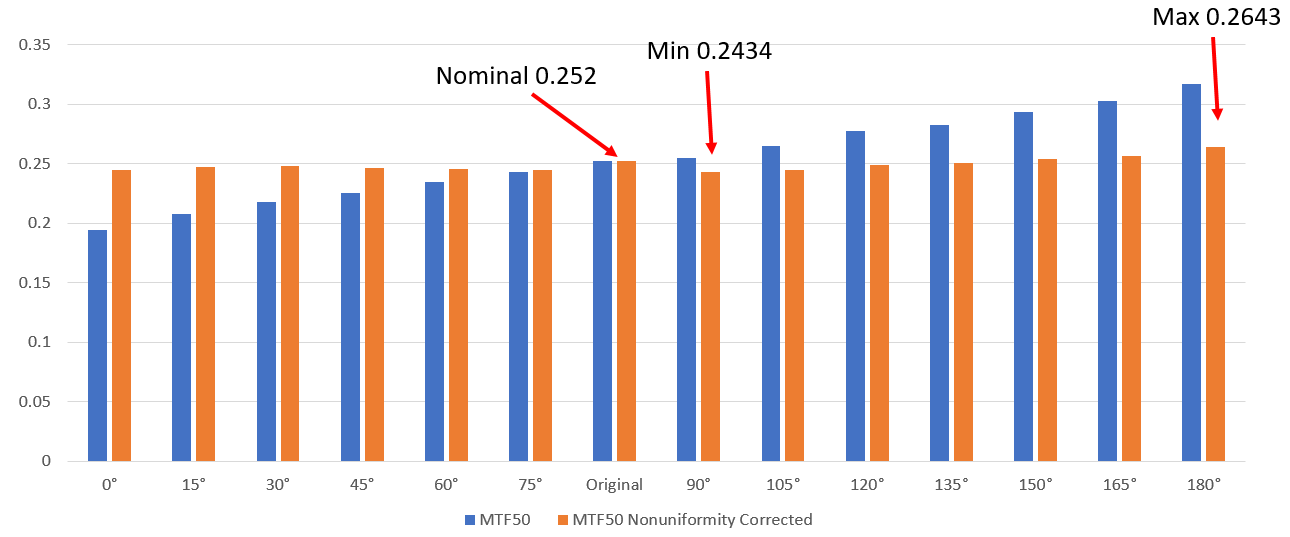MTF50 versus nonuniformity angle without [blue] and with [orange] nonuniformity correction

While this is a large improvement, the residual effects of nonuniformity remain undesirable. Because of this, we recommend turning on your ISP’s nonuniformity correction before performing edge-SFR tests or averaging the MTF obtained from nearby slanted edges with opposite transition directions relative to the nonuniformity to reduce the effects of nonuniformity on your MTF measurements further.

### Detailed algorithm

We assume that the illumination of the chart in the Region of Interest (ROI) approximates a first-order function, L(d) = k1 + k2d, where d is the horizontal or vertical distance nearly perpendicular to the (slanted) edge. The procedure consists of estimating k1 and k2, then dividing the linearized average edge by L(d).

k1 and k2, are estimated using the light side of the transition starting at a sufficient distance dN from the transition center xcenter, so the transition itself does not have much effect on the k1 and k2 estimate. To find dN we first find the 20% width d20 of the line spread function (LSF; the derivative of the edge), i.e., the distance between the points where the LSF falls to 20% of its maximum value.

dN = xcenter + 2 d20

If the edge response for x > dN has a sufficient number of points, it is used to calculate k1 and k2 using standard polynomial fitting techniques. The result is a more accurate representation of the edge with the effects of nonuniformity reduced.

### Future work

• Consider the 2D nonuniformity across the ROI before sampling the 1D average edge
• Use an image of a flat-field to perform nonuniformity correction within Imatest
• Consider the impact of noise which was not included in this study
• Incorporate enhancements to the slanted-edge algorithms into future revisions of ISO 12233

# Measuring temporal noise

Temporal noise is random noise that varies independently from image to image, in contrast to fixed-pattern noise, which remains consistent (but may be difficult to measure because it is usually much weaker than temporal noise). It can be analyzed by Colorcheck and Stepchart and was added to Multicharts and Multitest in Imatest 5.1 (Known as Color/Tone starting in Imatest 5.2)

It can be calculated by two methods.

1. the difference between two identical test chart images (the Imatest recommended method), and

2. the ISO 15739-based method, which where it is calculated from the pixel difference between the average of N identical images (N ≥ 8) and each individual image.

In this post we compare the two methods and show why method 1 is preferred.

(1) Two-image difference method. In any of the modules, read two images. The window shown on the right appears. Select the Read two files for measuring temporal noise radio button.

The two files will be read and their difference (which cancels fixed pattern noise) is taken. Since these images are independent, noise powers add. For indendent images I1 and I2, temporal noise is

$$\displaystyle \sigma_{temporal} = \frac{\sigma(I_1 – I_2)}{\sqrt{2}}$$

In Multicharts and Multitest temporal noise is displayed as dotted lines in Noise analysis plots 1-3 (simple noise, S/N, and SNR (dB)).

(2) Multiple image method. From ISO 15739, sections 6.2.4, 6.2.5, and Appendix A.1.4. Available in  Multicharts and Multitest. Currently we are using simple noise (not yet scene-referred noise). Select between 4 and 16 files. In the multi-image file list window (shown above) select Read n files for temporal noise. Temporal noise is calculated for each pixel j using

$$\displaystyle \sigma_{diff}(j) = \sqrt{ \frac{1}{N} \sum_{i=1}^N (X_{j,i} – X_{AVG,j})^2} = \sqrt{ \frac{1}{N} \sum_{i=1}^N X_{j,i}^2 – \left(\frac{1}{N} \sum_{i=1}^N X_{j,i}\right)^2 }$$

The latter expression is used in the actual calculation since only two arrays, $$\sum X_{j,i} \text{ and } \sum X_{j,i}^2$$, need to be saved. Since N is a relatively small number (between 4 and 16, with 8 recommended), it must be corrected using formulas for sample standard deviation from Identities and mathematical properties in the Wikipedia standard deviation page as well as Equation (13) from ISO 15739.  $$s(X) = \sqrt{\frac{N}{N-1}} \sqrt{E[(X – E(X))^2]}$$.

$$\sigma_{temporal} = \sigma_{diff} \sqrt{\frac{N}{N-1}}$$

We recommend the difference method (1)  when only the magnitude of temporal noise is required. Method (2), which requires many more images (≥ 8 recommended), allows fixed pattern noise and the noise image to be calculated at the same time.

To calculate temporal noise with either method, read the appropriate number of files (2 or ≥4) then push the appropriate radio button on the multi-image settings box.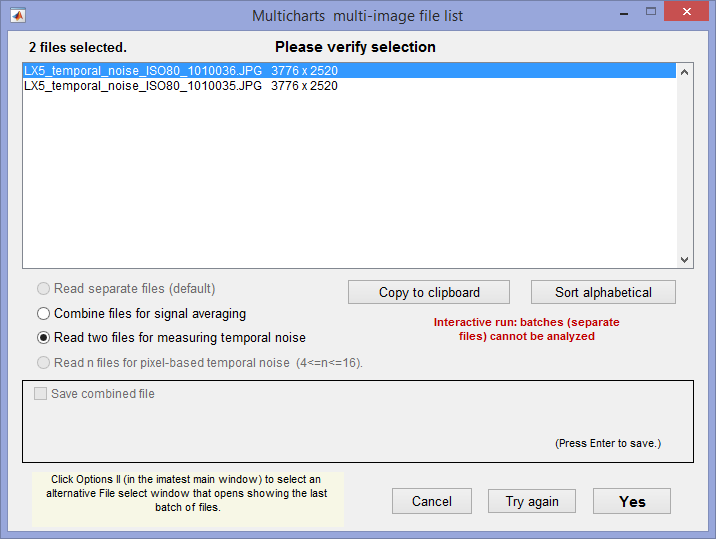Multi-image settings window, showing setting for method 1 (two-image difference).
For ≥4 images (multi-image method), select  • Read n files for … temporal noise, which will be enabled.

### Results for the two methods

The two methods were compared using identical Colorchecker images taken on a Panasonic Lumix LX5 camera (a moderately high quality small-sensor camera now several years old).

#### Difference method (1) (two files)

Here are the Multicharts results for 2 files.Multicharts SNR results for temporal noise, shown as thin dotted lines in the lower plot

#### Multi-file method (2) (4-16 files)

 Here are results (SNR (dB)) for runs with 4, 8, and 16 files. For 4 files, temporal SNR (thin dotted lines) is slightly better than standard noise. Temporal SNR is slightly lower for 8 files and very slightly lower for 16 files. For 8 and 16 files results are closer to the results for 2 files (though differences between 8 and 16 files are very small). The bottom line: We recommend the two-file (difference) method because it is accurate and relatively fast. The multi file method is slower for acquiring and analyzing images— at least 8 images are recommended, so why bother?Temporal SNR from 4 images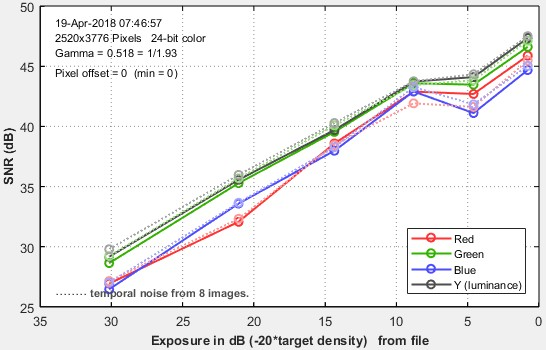Temporal SNR from 8 imagesTempoal SNR from 16 images

### Temporal noise image

 The full Electronic Imaging paper on the noise image can be found on Using images of noise to estimate image processing behavior for image quality evaluation. Noise can be measured anywhere in an image– on edges, etc.– if multiple identical images are acquired. This will lead to some interesting applications.

As we discussed in Uniformity statistics based on EMVA 1288, temporal noise σdiff (j), which is defined for each pixel j, can be displayed as an image. In order for the image to have good enough quality to display, more samples are required than for method (2) (above), which is used to calculate the average temporal noise in a patch — much less demanding than displaying an image. 32 is a reasonable minimum number of samples. 100 or 128 is even better.

Although temporal noise is measured using the same technique as EMVA 1288, there is an important difference. Any arbitrary image (test charts, natural scenes, etc.) can be used; not just flat-field images. This can provide insight into the behavior of image processing over the image — which can be valuable for bilateral filtered images, where the image processing, hence noise, varies over the image surface.

To obtain a temporal noise image, multiple images (typically at least 32) must be signal-averaged. This can be done by combining multiple image files or through direct read (more efficient if it’s available). The method for obtaining noise image is described in detail here. Uniformity Interactive is recommended for displaying temporal noise images. We review the key points.

 For direct data acquisition, make sure the camera and Device Manager are set to correctly capture the image of interest. The Preview has to be turned off to enable the adjustments. Click Save when the image in the Device Manager is correct.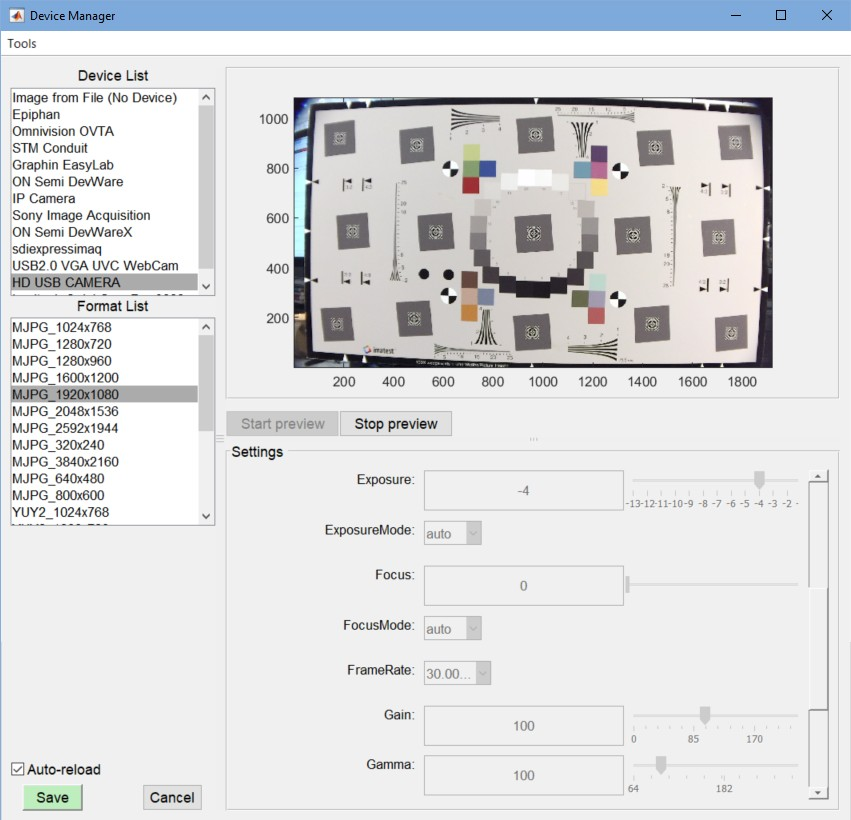In the Uniformity Interactive window, set Signal averaging to a large number (128 reads here), and check Calculate image^2 while averaging. Because of the sequence of operations in Uniformity Interactive, you may need to read an image (before you have the correct settings), then make the settings, then reread. You may want to crop the image when you read it to make it easier to examine specific regions of interest.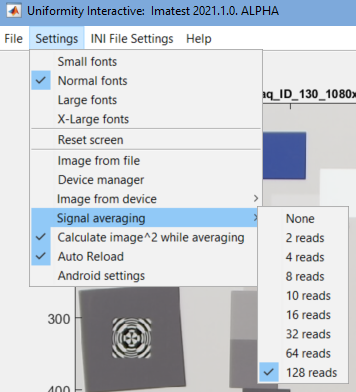Here is the original image (cropped). This image is virtually noiseless because the L = 128 averages increases the SNR (Signal-to-Noise Ratio) by 21 dB (3*log2(L)).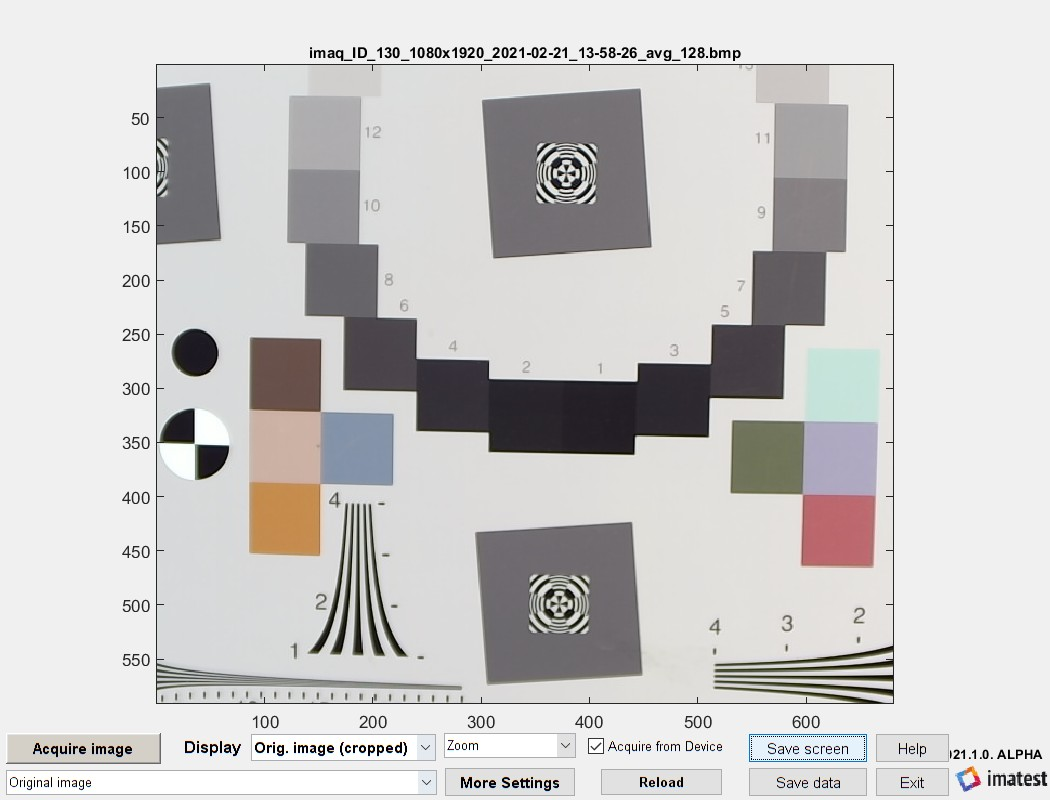Here is the noise image displayed auto-lightened. This gives a good picture of the noise, but lacks quantitative information. As expected, noise is largest near sharp edges and low in smooth areas of the chart. There is no noise in the white part of the registration mark because it’s fully saturated (pure white).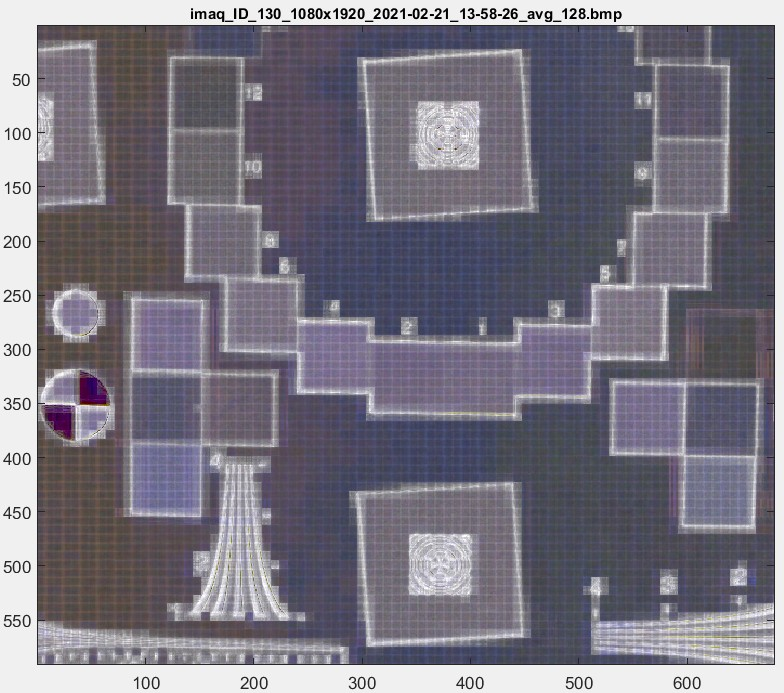Here is the noise image displayed in pseudocolor, which a numeric scale on the right.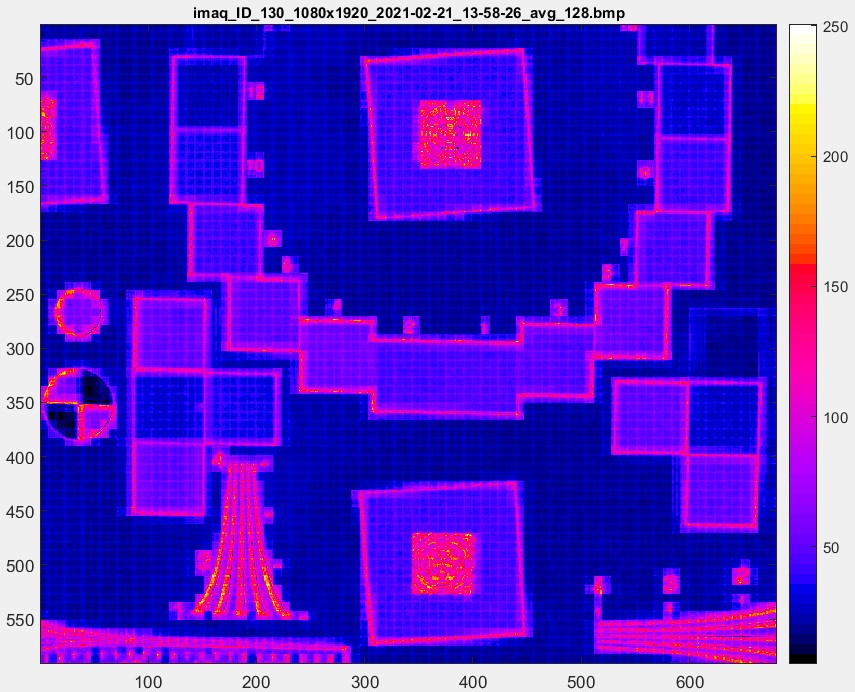Finally, here is the pseudocolor image greatly enlarged. The 8×8 pixel JPEG artifacts, characteristic of medium-low quality JPEG compression, are plainly visible.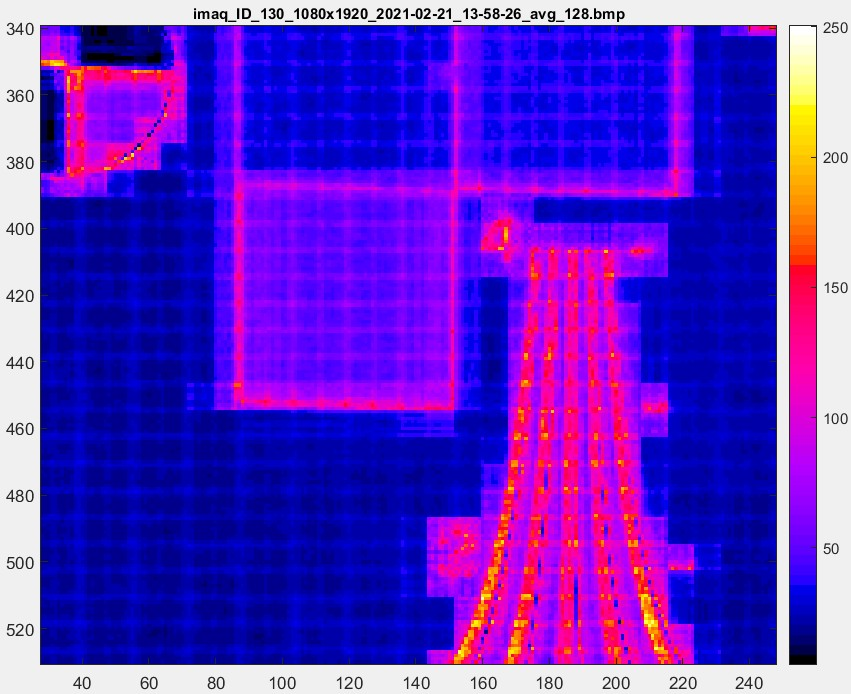# Color difference ellipses

Imatest has several two-dimensional displays for comparing test chart reference (ideal) colors with measured (camera) colors, where reference colors are represented by squares and measured values are represented by circles. (more…)

# SFRreg: SFR from Registration Marks

Imatest SFRreg performs highly automated measurements of sharpness (expressed as Spatial Frequency Response (SFR), also known as Modulation Transfer Function (MTF)) and Lateral Chromatic Aberration from images that contain registration mark patterns (circles with two light and two dark quadrants). Unlike standard test charts, these patterns do not need to be located on a flat (planar) surface. Depending on the image source, they offer two advantages. You can

 Test images at infinity distance (or any distance of choice) using a compact projection system such as the Optikos Meridian camera test system. Test the sharpness of extreme fisheye lenses (with angular fields of view over 180 degrees, whose MTF cannot be measured near the image boundaries with a single flat target) using an array test charts, each consisting of an individual registration mark. Registration mark charts, such as the one shown on the right, may be purchased from the Imatest store or printed on a high-quality inkjet printer. Since Region of Interest (ROI) selection is automatic, they may be positioned where needed. They work best when facing the camera.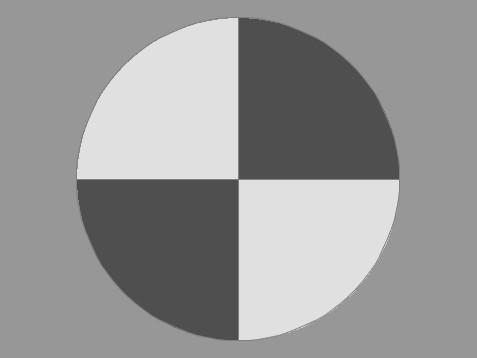Registration mark

Details of the regions to be analyzed are based on user-entered criteria (similar to SFRplus or eSFR ISO, which it closely resembles).

Sharpness is derived from light/dark slanted edges inside the registration marks, as described in Sharpness: What is it and how is it measured? SFRreg can handle a wide range of camera aspect ratios and chart arrangements.

SFRreg operates in two modes.

• Interactive/setup mode  allows you to select settings and interactively examine results in detail. Saved settings are used for Auto Mode.
• Auto mode  runs automatically with no additional user input. ROIs are located automatically based on settings saved from the interactive/setup mode. This allows images of different sizes and framing to be analyzed with no change of settings. Auto mode works with large batches of files, and is especially useful for automated testing, where framing may vary from image to image.

Part 1 of the instructions introduces SFRreg and explains how to obtain and photograph the chart. Part 2 shows how to run SFRreg inside Rescharts and how to save settings for automated runs. Part 3 illustrates the results.

### SFRreg images from the Optikos Meridian projection system

Imatest SFRreg is originally designed designed to work with images from the Optikos Meridian system, which consists of several projectors that project registration mark patterns towards a camera. These patterns appear at infinity focus at the camera. A typical image is shown below.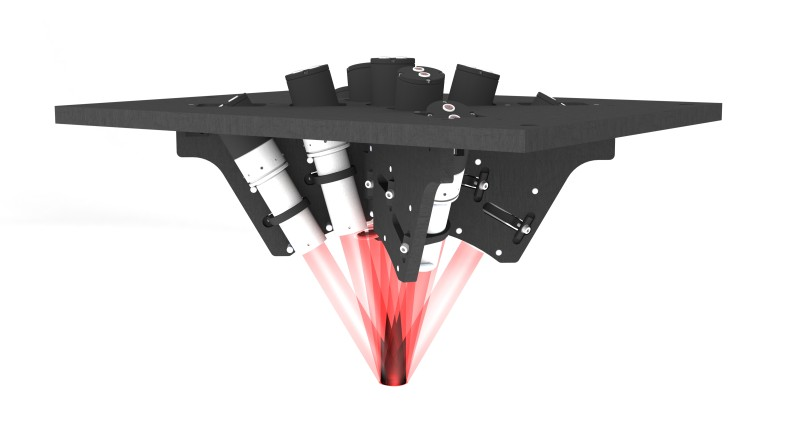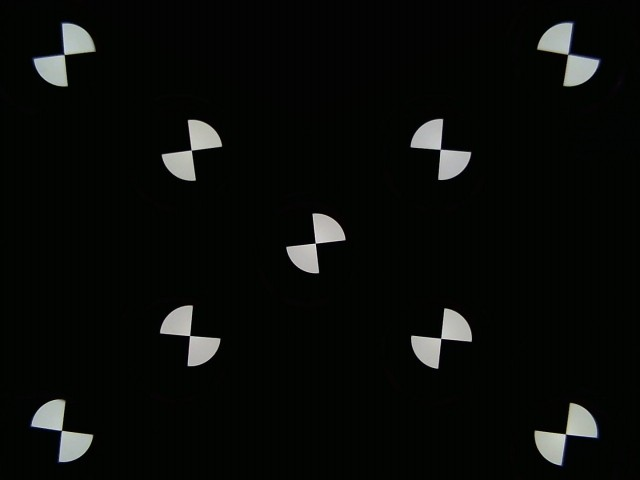Image acquired from a 9-projector Optikos Meridian system

### SFRreg images from arbitrary arrays of printed registration mark charts

SFRreg also works with printed registration mark patterns, which can be placed anywhere in the image. For extreme wide-angle (fisheye) lenses they should be oriented facing directly towards the camera. Here is a synthesized image (we’ll add a real one soon). You can add other charts— typically color or grayscale— to the image for additional measurements.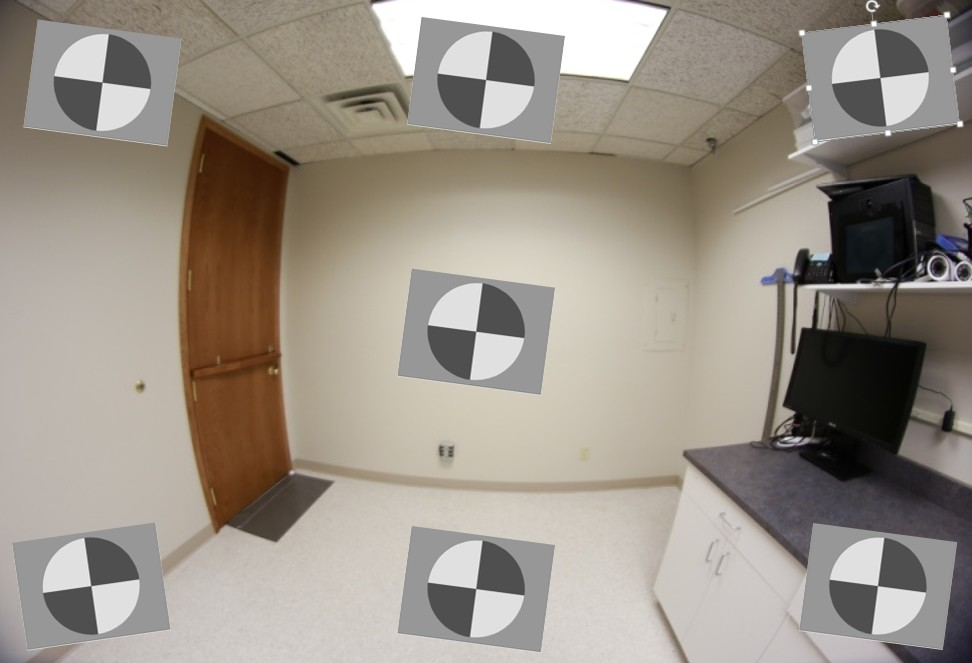Fisheye lens image with synthesized registration mark charts oriented facing the camera

SFRreg chart print options (can be selected when ordering)

Options Notes
Media Inkjet (reflective),
LVT film (transmissive)
Inkjet (reflective) is usually the most practical choice.
Contrast
4:1, 10:1
4:1 contrast is specified in the new ISO 12233:2014 standard.
Surface Matte or semigloss Semigloss is slightly sharper, but is more susceptible to glare (specular reflections), especially with wide angle lenses. Matte surface is recommended for wide angle lenses or difficult lighting situations.

 Slanted-edge algorithm The algorithms for calculating MTF/SFR were adapted from a Matlab program, sfrmat, written by Peter Burns (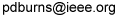) to implement the ISO 12233:2000 standard. Imatest SFR, SFRplus, SFRreg, and eSFR ISO incorporates numerous improvements, including improved edge detection, better handling of lens distortion, a nicer interface, and far more detailed output. The original Matlab code is available on http://losburns.com/imaging/software/SFRedge/index.htm. In comparing sfrmat results with Imatest, note that if no OECF (tonal response curve) file is entered into sfrmat, no tonal response curve is assumed, i.e., gamma = 1 (linear response). Since the default value of gamma in Imatest is 0.5, which is typical of digital cameras, you must set gamma to 1 to obtain good agreement with sfrmat.

#### Obtaining and photographing the charts

Registration Mark charts can be purchased from the Imatest store in a variety of inkjet media (reflective and transmissive) (Other media will be available on request.) Although we recommend that you purchase the charts, they can be printed on photographic-quality inkjet printers, but you must have fine materials, skill, and a knowledge of color management.

## SFRreg results

When calculations are complete, results are displayed in the Rescharts window, which allows a number of displays to be selected. The following table shows where specific results are displayed. Results plots are very similar to SFRplus and eSFR ISO. We show two samples of results below.

 Measurement Display MTF (sharpness) for individual regions 1. Edge and MTF MTF (sharpness) for entire image 4. Multi-ROI summary 12. 3D plot 13. Lens-style MTF plot Lateral Chromatic Aberration 2. Chromatic Aberration Original image showing region selection 8. Image & geometry EXIF data 7. Summary & EXIF data Acutance/SQF (Subjective Quality Factor) 3. SQF / Acutance Edge roughness 14. Edge roughness Chromatic Aberration (radial) 15. Radial (Chr Aber, etc.)

## Multi-ROI summary display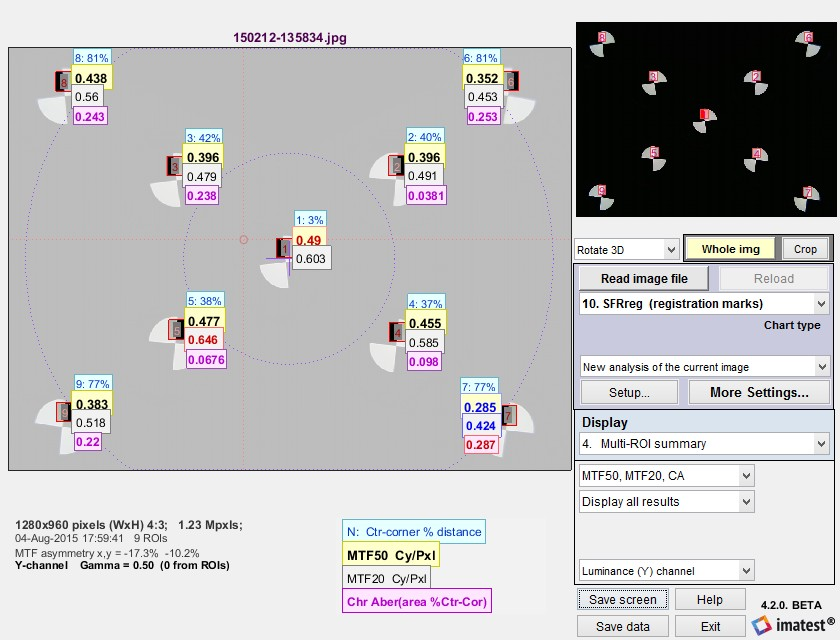SFRreg results in Rescharts window: Multiple region (ROI) summary
(Only upper Vertical regions have been selected to keep the view uncluttered.)

The multi-ROI (multiple Region of Interest) summary shown in the Rescharts window (above) contains a detailed summary of SFRreg results. (3D plots also contain an excellent summary.) The upper left contains the image in muted gray tones, with the selected regions surrounded by red rectangles and displayed with full contrast. Up to four results boxes are displayed next to each region. The results are selected the the Display options area on the right of the window, below the Display selection.

 The Results selection (right) lets you choose which results to display. N is region number. Ctr-corner distance % is the approximate location of the region. CA is Chromatic Aberration in area, as percentage of the center-to-corner distance (a perceptual measurement). A legend below the image shows which results are displayed. The View selection (far right) lets you select how many results boxes to display, which can be helpful when many regions overlap. From top to bottom the number of boxes is 4, 3, 2, 2, and 1, respectively.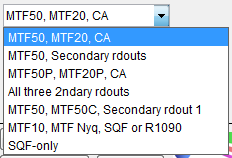Results selection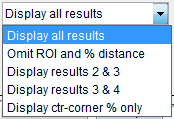View selection

## Edge and MTF display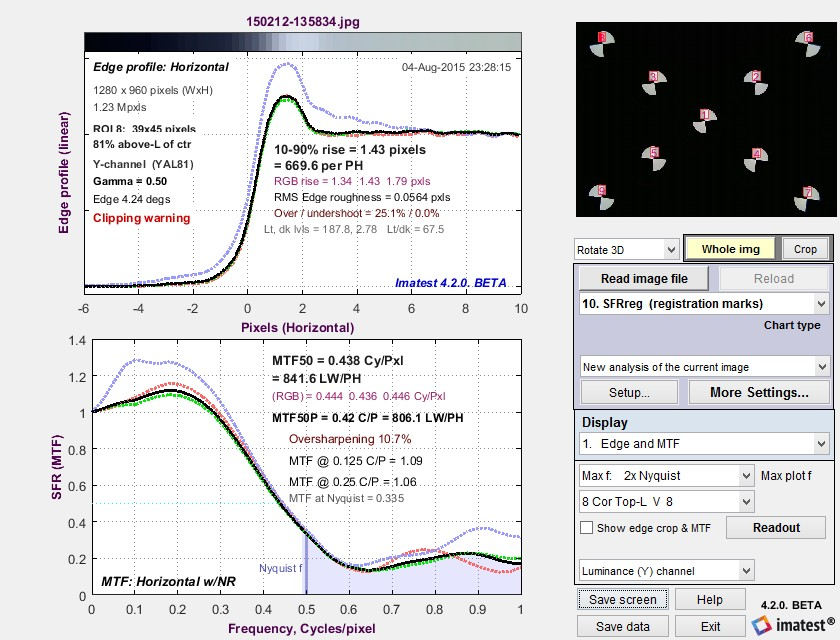Edge and MTF display in Rescharts window
Diffraction-limited MTF and edge response are shown as a pale brown dotted lines
when pixel spacing (5.7um for the EOS-40D) has been entered.

This display is identical to the SFR Edge and MTF display. The edge (or line spread function) is plotted on the top and the MTF is plotted on the bottom. The edge may be displayed linearized and normalized (the default; shown), unlinearized (pixel level) and normalized, or linearized and unnormalized (good for checking for saturation, especially in images with poor white balance). Edge display is selected by pressing .

There are a number of readouts, including 10-90% rise distance, MTF50, MTF50P (the spatial frequency where MTF is 50% of the peak value, differing from MTF50 only for oversharpened pulses), the secondary readouts (MTF @ 0.125 and 0.25 C/P in this case), and the MTF at the Nyquist frequency (0.5 cycles/pixel). The diffraction-limited MTF curve (not shown above) is displayed as a pale brown dotted line when pixel pitch is entered.

MTF is explained in Sharpness: What is it and how is it measured? MTF curves and Image appearance contains several examples illustrating the correlation between MTF curves and perceived sharpness.

# Measuring Multiburst pattern MTF with Stepchart

Measuring MTF is not a typical application for Stepchart— certainly not its primary function— but it can be useful with multiburst patterns, which are a legacy from analog imaging that occasionally appear in the digital world. The multiburst pattern is not one of Imatest’s preferred methods for measuring MTF: see the MTF Measurement Matrix for a concise list. But sometimes customers need to analyze them. This feature is available starting with Imatest 4.1.3 (March 2015).

# LSF correction factor for slanted-edge MTF measurements

A correction factor for the slanted-edge MTF (Edge SFR; E-SFR) calculations in SFR, SFRplus, eSFR ISO, SFRreg, and Checkerboard was added to Imatest in 2015. This correction factor is included in the ISO 12233:2014 and 2017 standards, but is not in the older ISO 12233:2000 standard. Because it corrects for an MTF loss caused by the numerical calculation of the Line Spread Function (LSF) from the Edge Spread Function (ESF), we call it the LSF correction factor. (more…)

# Slanted-Edge versus Siemens Star: A comparison of sensitivity to signal processing

This post addresses concerns about the sensitivity of slanted-edge patterns to signal processing, especially sharpening, and corrects the misconception that sinusoidal patterns, such as the Siemens star (included in the ISO 12233:2014 standard), are insensitive to sharpening, and hence provide more robust and stable MTF measurements. (more…)

# Sharpness and Texture Analysis using Log F‑Contrast from Imaging-Resource

Imaging-resource.com publishes images of the Imatest Log F-Contrast* chart in its excellent camera reviews. These images contain valuable information about camera quality— how sharpness and texture response are affected by image processing— but they need to be processed by Imatest to reveal the important information they contain.

*F is an abbreviation for Frequency in Log F-Contrast.

# Measuring Test Chart Patches with a Spectrophotometer

Using Babelcolor Patch Tool or SpectraShop 4

This post describes how to measure color and grayscale patches on a variety of test charts, including Imatest SFRplus and eSFR ISO charts, the X-Rite Colorchecker, ISO-15729, ISO-14524, ChromaDuMonde CDM-28R, and many more, using a spectrophotometer and one of two software packages.

# Region Selection bug workaround

Symptoms of problem:

Upon selection of a region of interest, program stops working, either not responding or crashing.

DOS window error messages:

• Operation terminated by user during pause (line 21) In newROI_quest
• Undefined function or method ‘figure1_KeyPressFcn’ for input arguments of type ‘struct’.
• Error while evaluating figure KeyPressFcn – Segmentation violation detected

Source of problem:

automatic translation software such as 有道首页 (Youdao Dictionary) and 金山词霸 (PowerWord)

Solution to problem:

Temporarily disable the translation software while performing a ROI selection

We will be working to make our software compatible with these sorts of translation programs in the future, as well as improving our own internationalization.  Sorry for the inconvenience.